# Typical Errors in Digital Audio: Part 3 – Aliasing

Reminder: This is still just the lead-up to the real topic of this series. However, we have to get some basics out of the way first…

In the first posting in this series, I talked about digital audio (more accurately, Linear Pulse Code Modulation or LPCM digital audio) is basically just a string of stored measurements of the electrical voltage that is analogous to the audio signal, which is a change in pressure over time… In the second posting in the series, we looked at a “trick” for dealing with the issue of quantisation (the fact that we have a limited resolution for measuring the amplitude of the audio signal). This trick is to add dither (a fancy word for “noise”) to the signal before we quantise it in order to randomise the error and turn it into noise instead of distortion.

In this posting, we’ll look at some of the problems incurred by the way we carve up time into discrete moments when we grab those samples.

Let’s make a wheel that has one spoke. We’ll rotate it at some speed, and make a film of it turning. We can define the rotational speed in RPM – rotations per minute, but this is not very useful. In this case, what’s more useful is to measure the wheel rotation speed in degrees per frame of the film.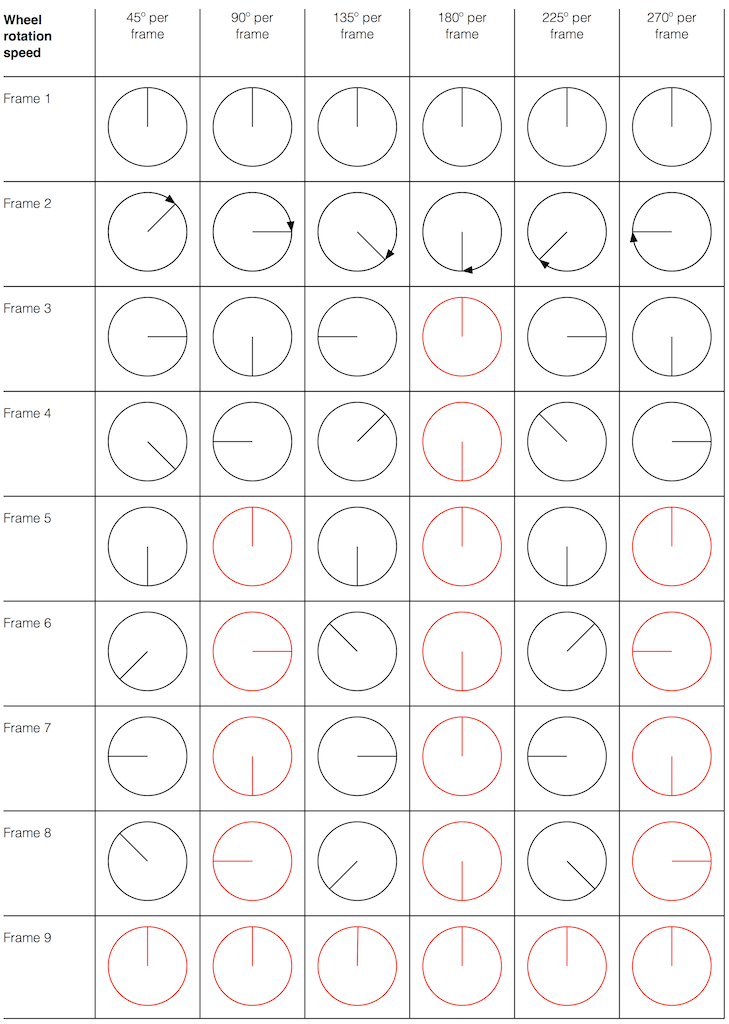Fig 1. The position of a clockwise-rotating wheel (with only one spoke) for 9 frames of a film. Each column shows a different rotational speed of the wheel. The far left column is the slowest rate of rotation. The far right column is the fastest rate of rotation. Red wheels show the frame in which the sequence starts repeating.

Take a look at the left-most column in Figure 1. This shows the wheel rotating 45º each frame. If we play back these frames, the wheel will look like it’s rotating 45º per frame. So, the playback of the wheel rotating looks the same as it does in real life.

This is more or less the same for the next two columns, showing rotational speeds of 90º and 135º per frame.

However, things change dramatically when we look at the next column – the wheel rotating at 180º per frame. Think about what this would look like if we played this movie (assuming that the frame rate is pretty fast – fast enough that we don’t see things blinking…) Instead of seeing a rotating wheel with only one spoke, we would see a wheel that’s not rotating – and with two spokes.

This is important, so let’s think about this some more. This means that, because we are cutting time into discrete moments (each frame is a “slice” of time) and at a regular rate (I’m assuming here that the frame rate of the film does not vary), then the movement of the wheel is recorded (since our 1 spoke turns into 2) but the direction of movement does not. (We don’t know whether the wheel is rotating clockwise or counter-clockwise. Both directions of rotation would result in the same film…)

Now, let’s move over one more column – where the wheel is rotating at 225º per frame. In this case, if we look at the film, it appears that the wheel is back to having only one spoke again – but it will appear to be rotating backwards at a rate of 135º per frame. So, although the wheel is rotating clockwise, the film shows it rotating counter-clockwise at a different (slower) speed. This is an effect that you’ve probably seen many times in films and on TV. What may come as a surprise is that this never happens in “real life” unless you’re in a place where the lights are flickering at a constant rate (as in the case of fluorescent or some LED lights, for example).

Again, we have to consider the fact that if the wheel actually were rotating counter-clockwise at 135º per frame, we would get exactly the same thing on the frames of the film as when the wheel if rotating clockwise at 225º per frame. These two events in real life will result in identical photos in the film. This is important – so if it didn’t make sense, read it again.

This means that, if all you know is what’s on the film, you cannot determine whether the wheel was going clockwise at 225º per frame, or counter-clockwise at 135º per frame. Both of these conclusions are valid interpretations of the “data” (the film). (Of course, there are more – the wheel could have rotated clockwise by 360º+225º = 585º or counter-clockwise by 360º+135º = 495º, for example…)

Since these two interpretations of reality are equally valid, we call the one we know is wrong an alias of the correct answer. If I say “The Big Apple”, most people will know that this is the same as saying “New York City” – it’s an alias that can be interpreted to mean the same thing.

We people in audio commit many sins. One of them is that, every time we draw a plot of anything called “audio” we start out by drawing a sine wave. (A similar sin is committed by musicians who, at the first opportunity to play a grand piano, will play a middle-C, as if there were other notes in the world.) The question is: what, exactly, is a sine wave?

Get a Slinky – or if you don’t want to spend money on a brand name, get a spring. Look at it from one end, and you’ll see that it’s a circle, as can be (sort of) seen in Figure 2.

Since this is a circle, we can put marks on the Slinky at various amounts of rotation, as in Figure 3.

Of course, I could have put the 0º marl anywhere. I could have also rotated counter-clockwise instead of clockwise. But since both of these are arbitrary choices, I’m not going to debate either one.

Now, let’s rotate the Slinky so that we’re looking at from the side. We’ll stretch it out a little too…

Let’s do that some more…

When you do this, and you look at the Slinky directly from one side, you are able to see the vertical change of the spring from the centre as a result of the change in rotation. For example, we can see in Figure 6 that, if you mark the 45º rotation point in this view, the distance from the centre of the spring is 71% of the maximum height of the spring (at 90º).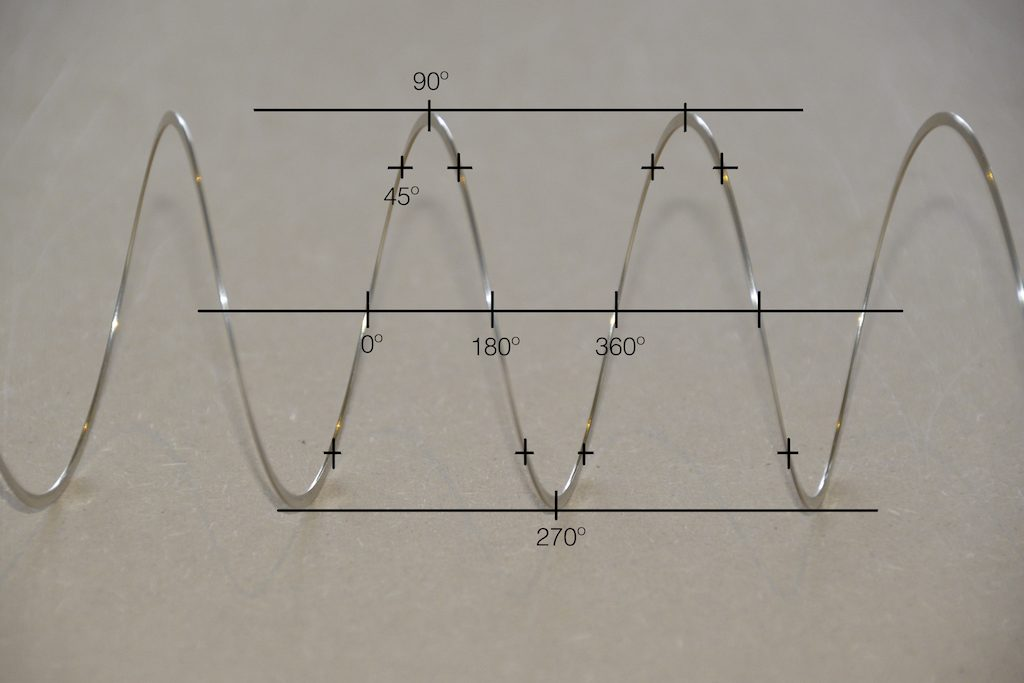Fig 6. The same markings shown in Figure 3, when looking at the Slinky from the side. Note that, if we didn’t have the advantage of a little perspective (and a spring made of flat metal), we would not know whether the 0º point was closer or further away from us than the 180º point. In other words, we wouldn’t know if the Slinky was rotating clockwise or counter-clockwise.

So what? Well, basically, the “punch line” here is that a sine wave is actually a “side view” of a rotation. So, Figure 7, shows a measurement – a capture – of the amplitude of the signal every 45º.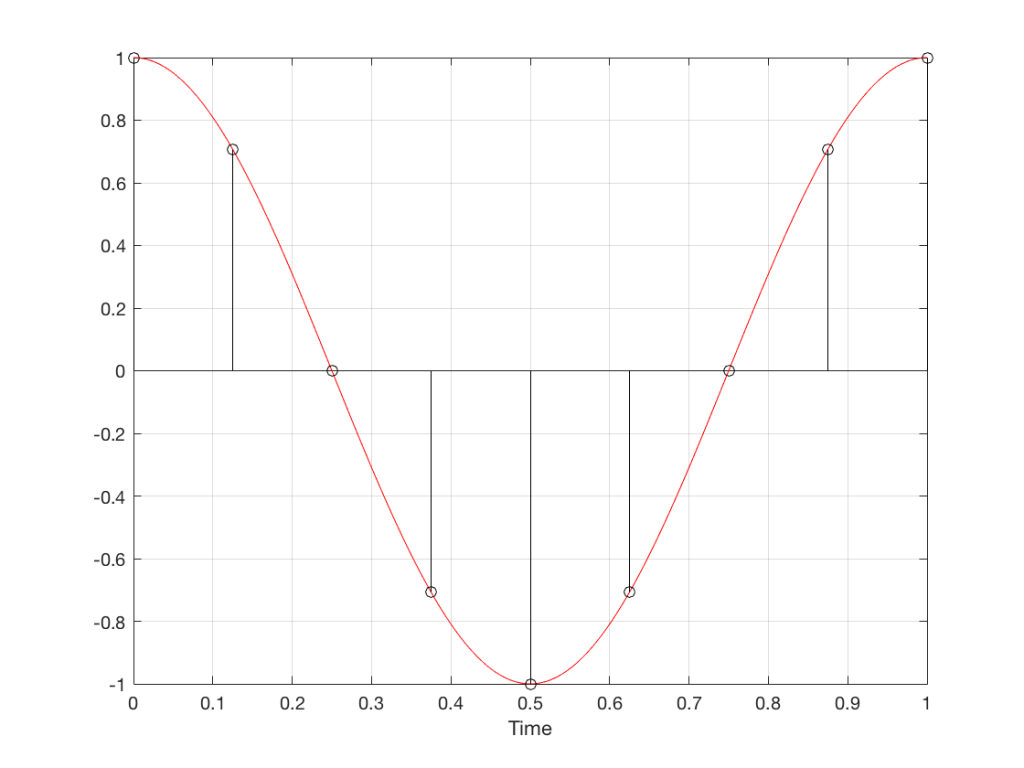Fig 7. Each measurement (a black “lollipop”) is a measurement of the vertical change of the signal as a result of rotating 45º.

Since we can now think of a sine wave as a rotation of a circle viewed from the side, it should be just a small leap to see that Figure 7 and the left-most column of Figure 1 are basically identical.

Let’s make audio equivalents of the different columns in Figure 1.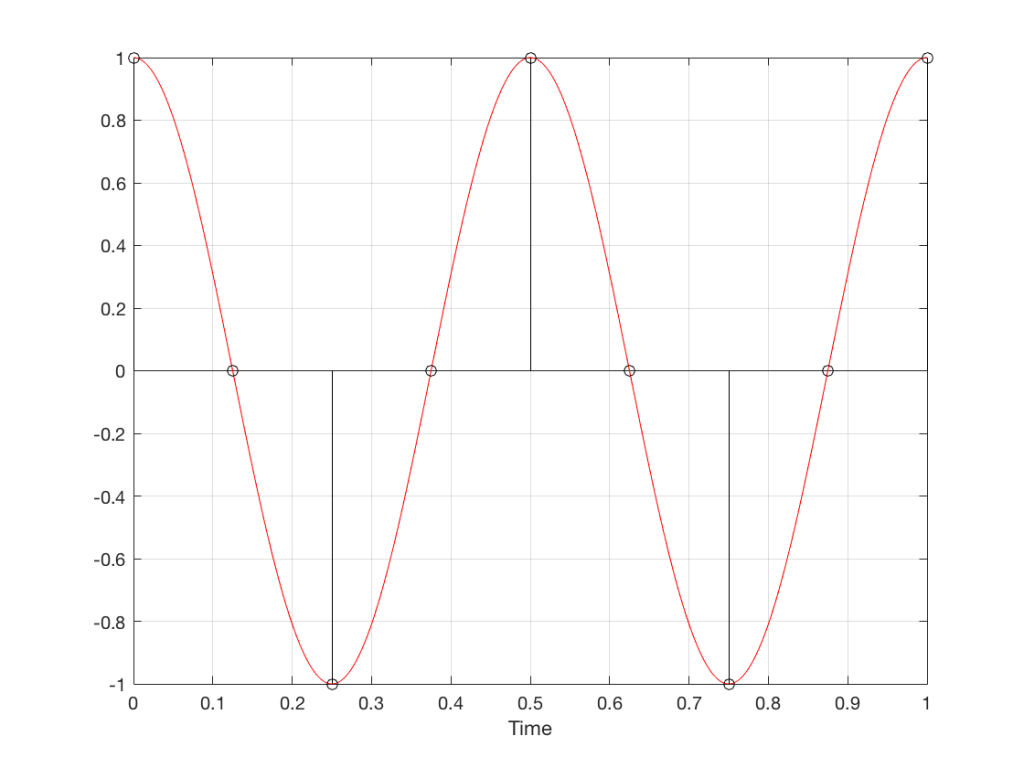Fig 8. A sampled cosine wave where the frequency of the signal is equivalent to 90º per sample period. This is identical to the “90º per frame” column in Figure 1.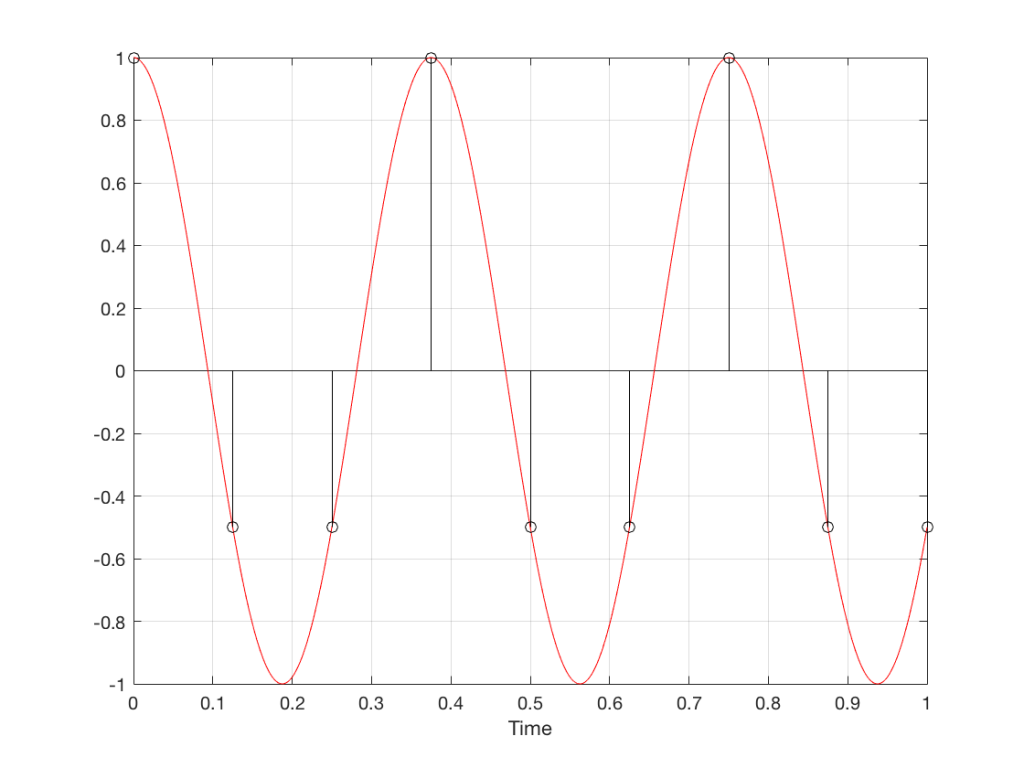Fig 9. A sampled cosine wave where the frequency of the signal is equivalent to 135º per sample period. This is identical to the “135º per frame” column in Figure 1.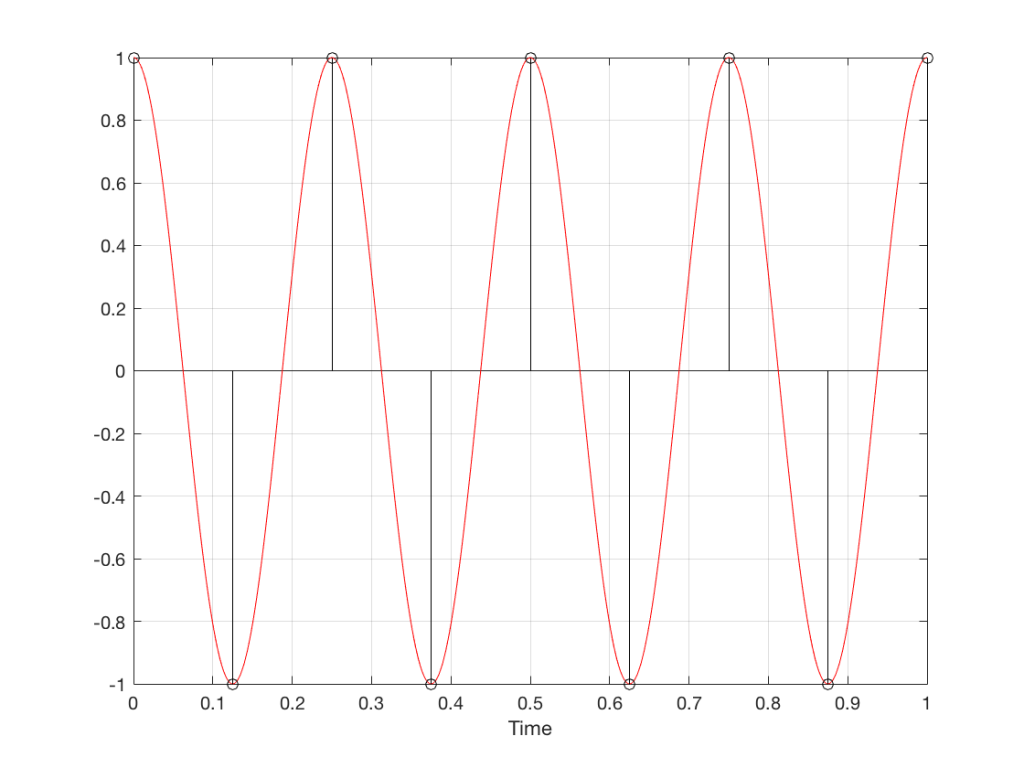Fig 10. A sampled cosine wave where the frequency of the signal is equivalent to 180º per sample period. This is identical to the “180º per frame” column in Figure 1.

Figure 10 is an important one. Notice that we have a case here where there are exactly 2 samples per period of the cosine wave. This means that our sampling frequency (the number of samples we make per second) is exactly one-half of the frequency of the signal. If the signal gets any higher in frequency than this, then we will be making fewer than 2 samples per period. And, as we saw in Figure 1, this is where things start to go haywire.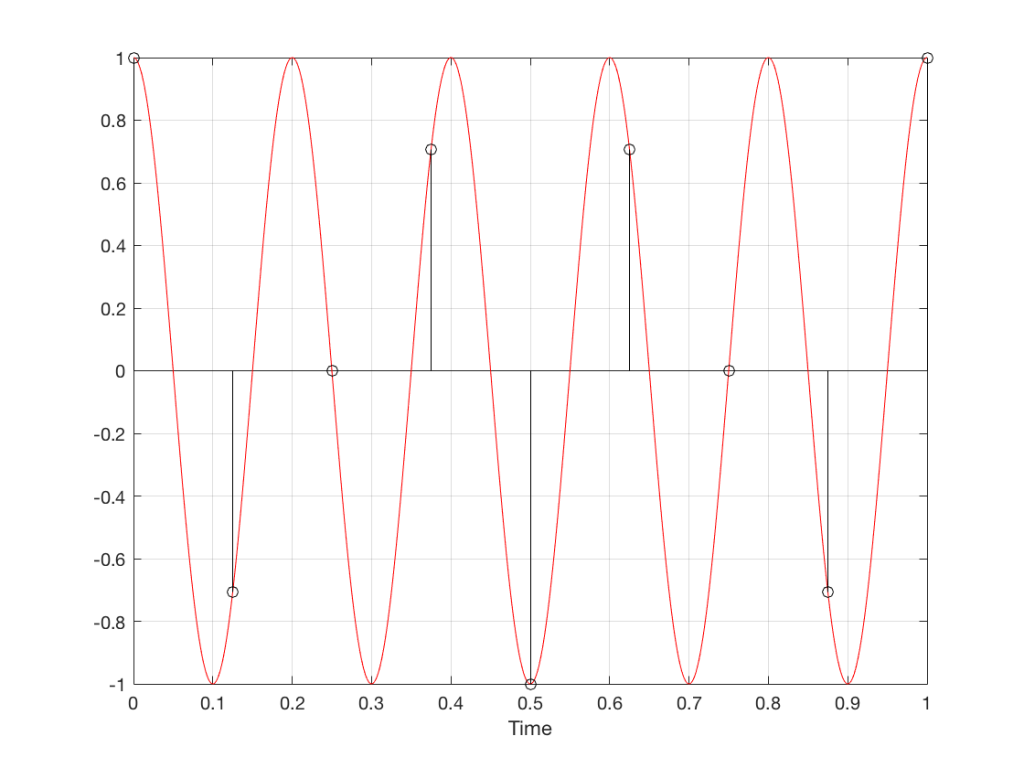Fig 11. A sampled cosine wave where the frequency of the signal is equivalent to 225º per sample period. This is identical to the “225º per frame” column in Figure 1.

Figure 11 shows the equivalent audio case to the “225º per frame” column in Figure 1. When we were talking about rotating wheels, we saw that this resulted in a film that looked like the wheel was rotating backwards at the wrong speed. The audio equivalent of this “wrong speed” is “a different frequency” – the alias of the actual frequency. However, we have to remember that both the correct frequency and the alias are valid answers – so, in fact, both frequencies (or, more accurately, all of the frequencies) exist in the signal.

So, we could take Fig 11, look at the samples (the black lollipops) and figure out what other frequency fits these. That’s shown in Figure 12.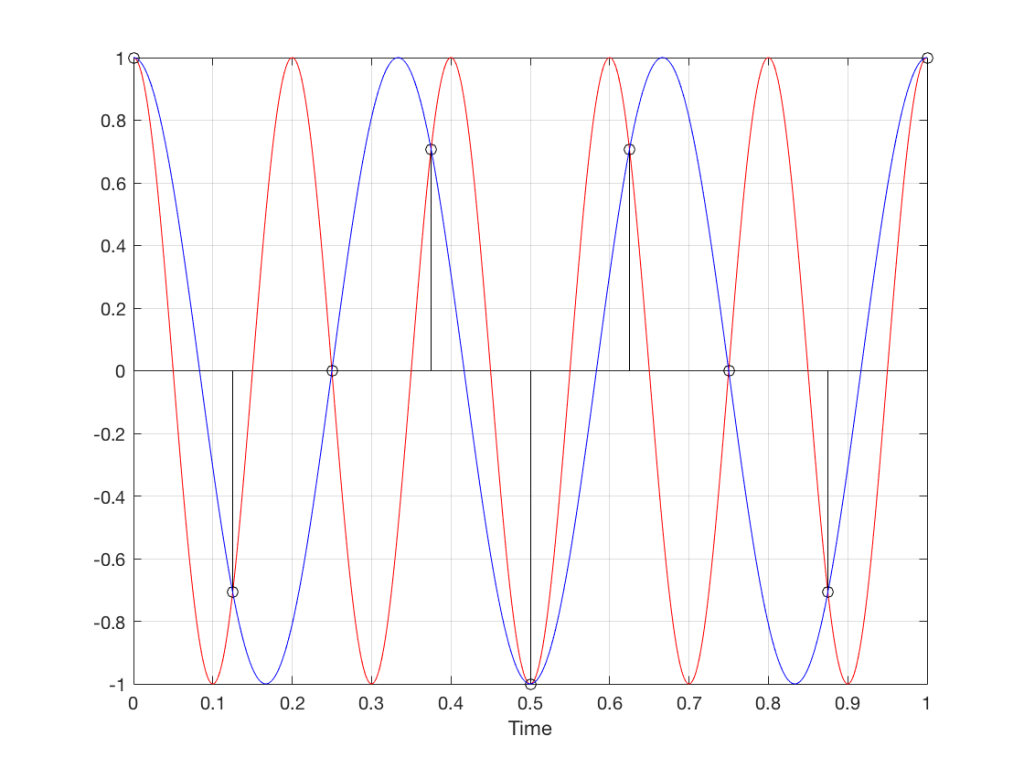Fig 12. The red signal and the black samples of it are the same as was shown in Figure 11. However, another frequency (the blue signal) also fits those samples. So, both the red signal and the blue signal exist in our system.

Moving up in frequency one more step, we get to the right-hand column in Figure 1, whose equivalent, including the aliased signal, are shown in Figure 13.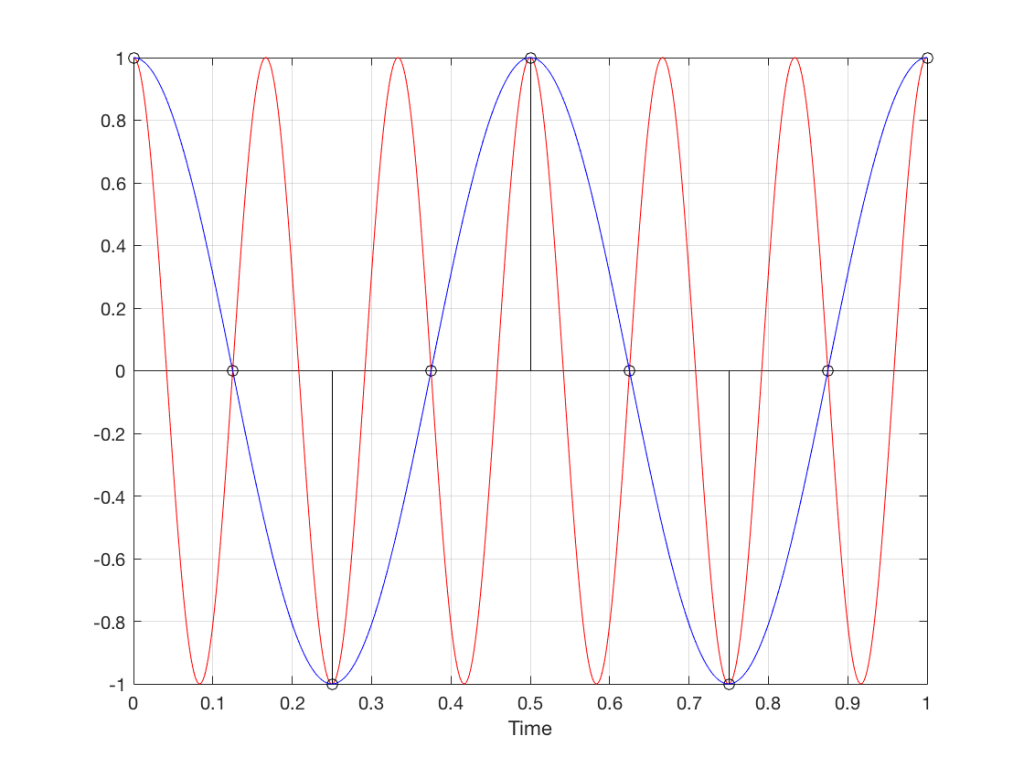Fig 13. A signal (the red curve) that has a frequency equivalent to 280º of rotation per sample, its samples (the black lollipops) and the aliased additional signal that results (the blue curve).

## Do I need to worry yet?

Hopefully, now, you can see that an LPCM system has a limit with respect to the maximum frequency that it can deal with appropriately. Specifically, the signal that you are trying to capture CANNOT exceed one-half of the sampling rate. So, if you are recording a CD, which has a sampling rate of 44,100 samples per second (or 44.1 kHz) then you CANNOT have any audio signals in that system that are higher than 22,050 Hz.

That limit is commonly known as the “Nyquist frequency“, named after Harry Nyquist – one of the persons who figured out that this limit exists.

In theory, this is always true. So, when someone did the recording destined for the CD, they made sure that the signal went through a low-pass filter that eliminated all signals above the Nyquist frequency.

In practice, however, there are many cases where aliasing occurs in digital audio systems because someone wasn’t paying enough attention to what was happening “under the hood” in the signal processing of an audio device. This will come up later.

## Two more details to remember…

There’s an easy way to predict the output of a system that’s suffering from aliasing if your input is sinusoidal (and therefore contains only one frequency). The frequency of the output signal will be the same distance from the Nyquist frequency as the frequency if the input signal. In other words, the Nyquist frequency is like a “mirror” that “reflects” the frequency of the input signal to another frequency below Nyquist.

This can be easily seen in the upper plot of Figure 14. The distance from the Input signal and the Nyquist is the same as the distance between the output signal and the Nyquist.

Also, since that Nyquist frequency acts as a mirror, then the Input and output signal’s frequencies will move in opposite directions (this point will help later).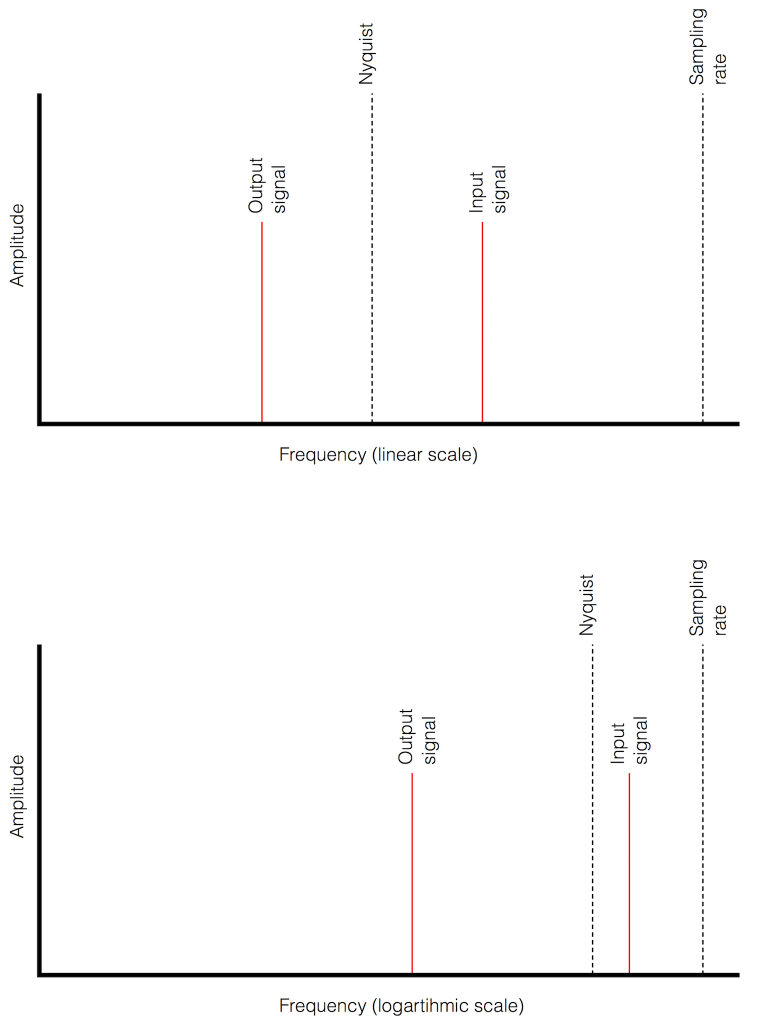Fig 14. Two plots showing the same information about an Input Signal above the Nyquist frequency and the output alias signal. Notice that, in the linear plot on top, it’s easier to see that the Nyquist frequency is the mirror point at the centre of the frequencies of the Input and Output signals.

Usually, frequency-domain plots are done on a logarithmic scale, because this is more intuitive for we humans who hear logarithmically. (For example, we hear two consecutive octaves on a piano as having the same “interval” or “width”. We don’t hear the width of the upper octave as being twice as wide, like a measurement system does. that’s why music notation does not get wider on the top, with a really tall treble clef.) This means that it’s not as obvious that the Nyquist frequency is in the centre of the frequencies of the input signal and its alias below Nyquist.

# Typical Errors in Digital Audio: Part 2 – Dither

Reminder: This is still just the lead-up to the real topic of this series. However, we have to get some basics out of the way first…

In the last posting, I talked about digital audio (more accurately, Linear Pulse Code Modulation or LPCM digital audio) is basically just a string of stored measurements of the electrical voltage that is analogous to the audio signal, which is a change in pressure over time…

For now, we’ll say that each measurement is rounded off to the nearest possible “tick” on the ruler that we’re using to measure the voltage. That rounding results in an error. However, (assuming that everything is working correctly) that error can never be bigger than 1/2 of a “step”. Therefore, in order to reduce the amount of error, we need to increase the number of ticks on the ruler.

Now we have to introduce a new word. If we really had a ruler, we could talk about whether the ticks are 1 mm apart – or 1/16″ – or whatever. We talk about the resolution of the ruler in terms of distance between ticks. However, if we are going to be more general, we can talk about the distance between two ticks being one “quantum” – a fancy word for the smallest step size on the ruler.

So, when you’re “rounding off to the nearest value” you are “quantising” the measurement (or “quantizing” it, if you live in Noah Webster’s country and therefore you harbor the belief that wordz should be spelled like they sound – and therefore the world needz more zees). This also means that the amount of error that you get as a result of that “rounding off” is called “quantisation error“.

In some explanations of this problem, you may read that this error is called “quantisation noise”. However, this isn’t always correct. This is because if something is “noise” then is is random, and therefore impossible to predict. However, that’s not strictly the case for quantisation error. If you know the signal, and you know the quantisation values, then you’ll be able to predict exactly what the error will be. So, although that error might sound like noise, technically speaking, it’s not. This can easily be seen in Figures 1 through 3 which demonstrate that the quantisation error causes a periodic, predictable error (and therefore harmonic distortion), not a random error (and therefore noise).

Sidebar: The reason people call it quantisation noise is that, if the signal is complicated (unlike a sine wave) and high in level relative to the quantisation levels – say a recording of Britney Spears, for example – then the distortion that is generated sounds “random-ish”, which causes people to just to the conclusion that it’s noise.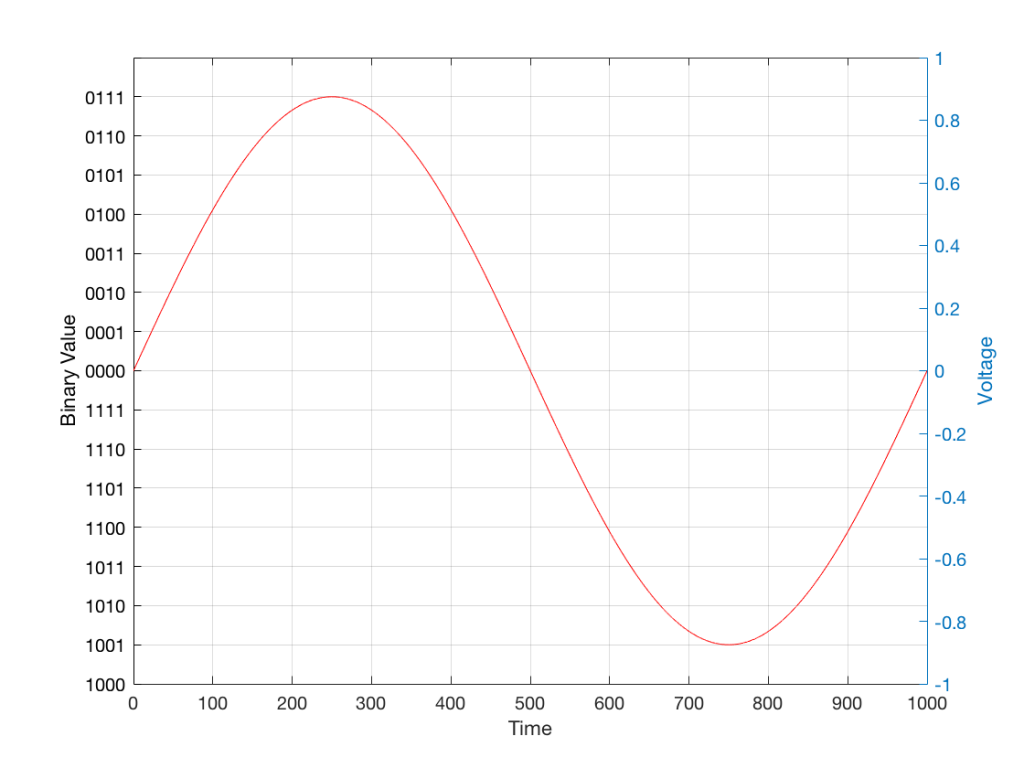Fig 1: The first cycle of a periodic signal (in this case, a sinusoidal waveform) that we are going to quantise using a 4-bit system (notice the 4 bits in the scale on the left).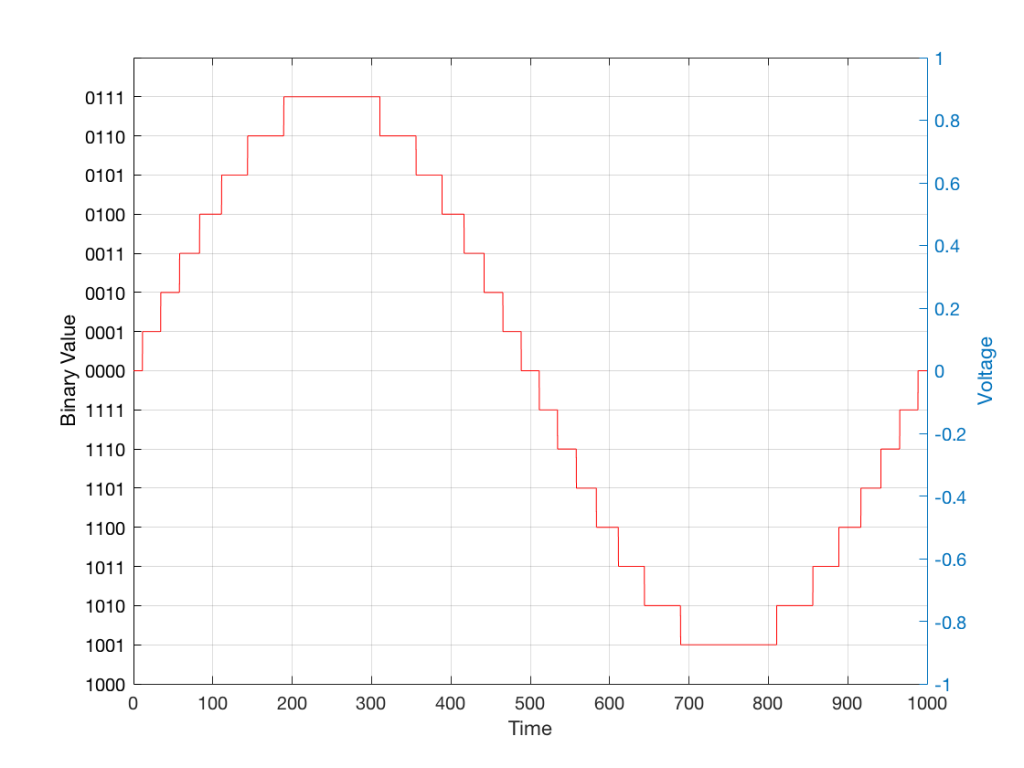Fig 2: The same waveform shown in Figure 1 after quantisation (rounding off) in a 4-bit world.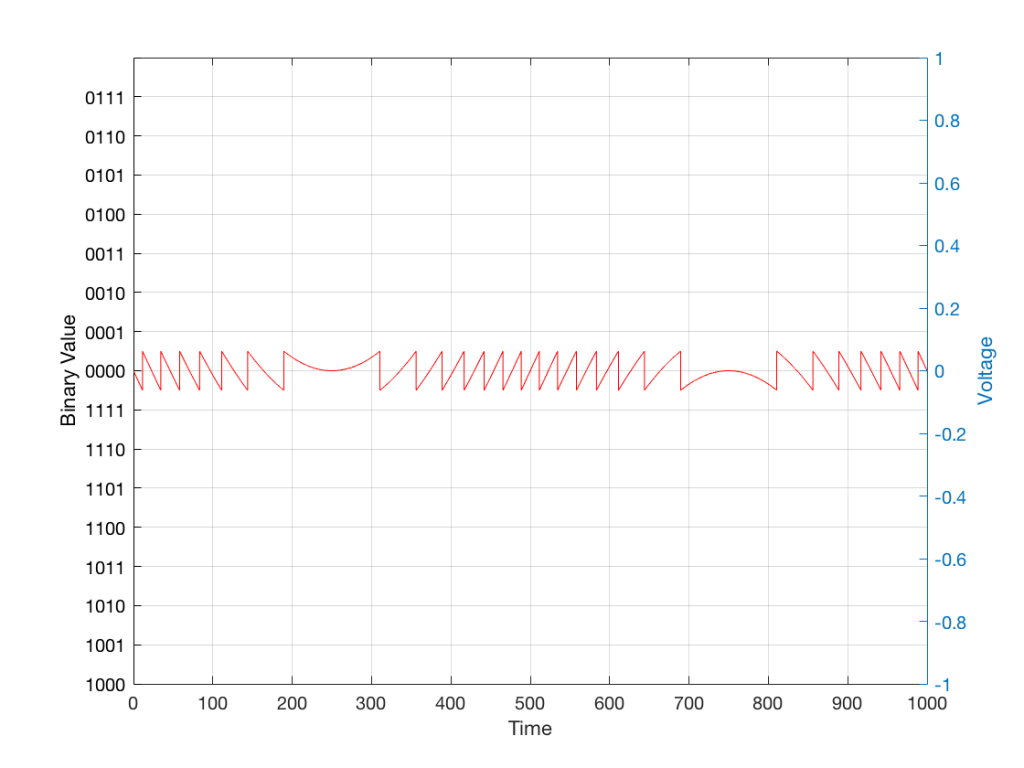Fig 3: The difference between Figure 2 and Figure 1. I made this by subtracting the original signal from the quantised version. This is the error in the quantised waveform – the quantisation error. Notice that it is not noise… it’s completely predictable and it will repeat with repetitions of the signal. Therefore the result of this is distortion, not noise…

Now, let’s talk about perception for a while… We humans are really good at detecting patterns – signals – in an otherwise noisy world. This is just as true with hearing as it is with vision. So, if you have a sound that exists in a truly random background noise, then you can focus on listening to the sound and ignore the noise. For example, if you (like me) are old enough to have used cassette tapes, then you can remember listening to songs with a high background noise (the “tape hiss”) – but it wasn’t too annoying because the hiss was independent of the music, and constant. However, if you, like me, have listened to Bob Marley’s live version of “No Woman No Cry” from the “Legend” album, then you, like me, would miss the the feedback in the PA system at that point in the song when the FoH engineer wasn’t paying enough attention… That noise (the howl of the feedback) is not noise – it’s a signal… Which makes it just as important as the song itself. (I could get into a long boring talk about John Cage at this point, but I’ll try to not get too distracted…)

The problem with the signal in Figure 2 is that the error (shown in Figure 3) is periodic – it’s a signal that demands attention. If the signal that I was sending into the quantisation system (in Figure 1) was a little more complicated than a sine wave – say a sine wave with an amplitude modulation – then the error would be easily “trackable” by anyone who was listening.

So, what we want to do is to quantise the signal (because we’re assuming that we can’t make a better “ruler”) but to make the error random – so it is changed from distortion to noise. We do this by adding noise to the signal before we quantise it. The result of this is that the error will be randomised, and will become independent of the original signal… So, instead of a modulating signal with modulated distortion, we get a modulated signal with constant noise – which is easier for us to ignore. (It has the added benefit of spreading the frequency content of the error over a wide frequency band, rather than being stuck on the harmonics of the original signal… but let’s not talk about that…)

## For example…

Let’s take a look at an example of this from an equivalent world – digital photography.

The photo in Figure 4 is a black and white photo – which actually means that it’s comprised of shades of gray ranging from black all the way to white. The photo has 272,640 individual pixels (because it’s 640 pixels wide and 426 pixels high). Each of those pixels is some shade of gray, but that shading does not have an infinite resolution. There are “only” 256 possible shades of gray available for each pixel.

So, each pixel has a number that can range from 0 (black) up to 255 (white).Fig 4: A photo of a building in Paris. Each pixel in this photo has one of 256 possible levels of gray – from white (255) down to black (0).

If we were to zoom in to the top left corner of the photo and look at the values of the 64 pixels there (an 8×8 pixel square), you’d see that they are:

86 86 90 88 87 87 90 91
86 88 90 90 89 87 90 91
88 89 91 90 89 89 90 94
88 90 91 93 90 90 93 94
89 93 94 94 91 93 94 96
90 93 94 95 94 91 95 96
93 94 97 95 94 95 96 97
93 94 97 97 96 94 97 97

What if we were to reduce the available resolution so that there were fewer shades of gray between white and black? We can take the photo in Figure 1 and round the value in each pixel to the new value. For example, Figure 5 shows an example of the same photo reduced to only 4 levels of gray.Fig 5: The same photo of the same building. Each pixel in this photo has one of 4 possible levels of gray – 255 (white), 170, 85 and 0 (black). Notice that some details are lost – like the smooth transitions in the clouds, or the stripes in the marble in the pillars.

Now, if we look at those same pixels in the upper left corner, we’d see that their values are

102 102 102 102 102 102 102 102
102 102 102 102 102 102 102 102
102 102 102 102 102 102 102 102
102 102 102 102 102 102 102 102
102 102 102 102 102 102 102 102
102 102 102 102 102 102 102 102
102 102 102 102 102 102 102 102
102 102 102 102 102 102 102 102

They’ve all been quantised to the nearest available level, which is 102. (Our possible values are restricted to 0, 51, 102, 154, 205, and 255).

So, we can see that, by quantising the gray levels from 256 possible values down to only 6, we lose details in the photo. This should not be a surprise… That loss of detail means that, for example, the gentle transition from lighter to darker gray in the sky in the original is “flattened” to a light spot in a darker background, with a jagged edge at the transition between the two. Also, the details of the wall pillars between the windows are lost.

If we take our original photo and add noise to it – so were adding a random value to the value of each pixel in the original photo (I won’t talk about the range of those random values…) it will look like Figure 6. This photo has all 256 possible values of gray – the same as in Figure 1.Fig 6: Noise. This “photo” has the same number of pixels (640 x 480) as the photo in Figure 4. We add this to the photo before asking the computer to reduce the number of “colours”.

If we then quantise Figure 6 using our 6 possible values of gray, we get Figure 7. Notice that, although we do not have more grays than in Figure 5, we can see things like the gradual shading in the sky and some details in the walls between the tall windows.Fig 7: The same photo of the same building in Figure 4. Each pixel in this photo ALSO only has one of 6 possible levels of gray – just like in Figure 5. However, this version is the result of quantising the original photo with the noise added before quantisation. The result is admittedly noisy – but we are able to see pattens in the noise that preserve some of the details that we lost in Figure 5.

That noise that we add to the original signal is called dither – because it is forcing the quantiser to be indecisive about which level to quantise to choose.

I should be clear here and say that dither does not eliminate quantisation error. The purpose of dither is to randomise the error, turning the quantisation error into noise instead of distortion. This makes it (among other things) independent of the signal that you’re listening to, so it’s easier for your brain to separate it from the music, and ignore it.

## Addendum: Binary basics and SNR

We normally write down our numbers using a “base 10” notation. So, when I write down 9374 – I mean
9 x 1000 + 3 x 100 + 7 x 10 + 4 x 1
or
9 x 103 + 3 x 102 + 7 x 101 + 4 x 100

We use base 10 notation – a system based on 10 digits (0 through 9) because we have 10 fingers.

If we only had 2 fingers, we would do things differently… We would only have 2 digits (0 and 1) and we would write down numbers like this:
11101

which would be the same as saying
1 x 16 + 1 x 8 + 1 x 4 + 0 x 2 + 1 x 1
or
1 x 24 + 1 x 23 + 1 x 22 + 0 x 21 + 1 x 20

The details of this are not important – but one small point is. If we’re using a base-10 system and we increase the number by one more digit – say, going from a 3-digit number to a 4-digit number, then we increase the possible number of values we can represent by a factor of 10. (in other words, there are 10 times as many possible values in the number XXXX than in XXX.)

If we’re using a base-2 system and we increase by one extra digit, we increase the number of possible values by a factor of 2. So XXXX has 2 times as many possible values as XXX.

Now, remember that the error that we generate when we quantise is no bigger than 1/2 of a quantisation step, regardless of the number of steps. So, if we double the number of steps (by adding an extra binary digit or bit to the value that we’re storing), then the signal can be twice as “far away” from the quantisation error.

This means that, by adding an extra bit to the stored value, we increase the potential signal-to-error ratio of our LPCM system by a factor of 2 – or 6.02 dB.

So, if we have a 16-bit LPCM signal, then a sine wave at the maximum level that it can be without clipping is about 6 dB/bit * 16 bits – 3 dB = 93 dB louder than the error. The reason we subtract the 3 dB from the value is that the error is +/- 0.5 of a quantisation step (normally called an “LSB” or “Least Significant Bit”).

Note as well that this calculation is just a rule of thumb. It is neither precise nor accurate, since the details of exactly what kind of error we have will have a minor effect on the actual number. However, it will be close enough.

# Typical Errors in Digital Audio: Part 1

## Introduction

Once upon a time, when I was a young whipper snapper, studying how to be a recording engineer (which is half of being a tonmeister) I had a textbook on sound recording. There were chapters in there on musical instruments, acoustics, microphones, mixing consoles, magnetic tape, and so on.. There was also a section on something called “digital audio” – but it was a portion of the chapter titled “Noise Reduction”.

Fast-forward a couple of years to 1983 and a new technology hit the market called “Compact Disc” (Here’s a fun fact for impressing people at your next dinner party: The “c” at the end of “disc” means it’s an optical medium. If it were magnetic, it would be a “disk”. So: Compact Disc, but Hard Disk.) Back then, the magazine advertisement read “Perfect Sound. Forever.” Then it hit the real world and the complaints started rolling in from people who believed that they knew things about audio. Some of these complaints were valid, and some were less so… Many of the ones that were valid no longer are, but it’s difficult to un-do a first impression.

Nowadays, it is very likely that almost-all-to-all of the music you listen to has been digital at some point in its life. Even if you’re listening to vinyl, it should not surprise you to know that the master version of the recording you’re hearing was probably stored on a hard disk or passed through a digital mixing console – or at least some of the tracks included some kind of digital processing (say, a guitar pedal or a reverb unit, for example). (I know, I know… There are exceptions. However, if you want to send me anti-digital hate mail you may not do it using a digital communication format such as e-mail. Use an analogue pen to write out your words on a piece of paper and send it to me by post. I look forward to receiving your analogue letters.)

Nowadays, a big part of my “day job” is to test (digital) audio systems to find out what’s wrong with them. So, I thought it would be interesting to do a series of postings that describe the typical kinds of errors that I look for (and find) when I’m digging down into the details.

In order to do this, I’m going to start by being a little redundant and describe the basics of how audio is converted from an analogue signal to a digital one – and hopefully address some of the misconceptions that are associated with this conversion process.

## A quick introduction to sound

At the simplest level, sound can be described as a small change in air pressure (or barometric pressure) over short periods of time. If you’d like to have a better and more edu-tain-y version of this statement with animations and pretty colours, you could take 10 minutes to watch this video, for example.

That change in pressure can be “captured” by using a microphone, that is (at the simplest level) a device that has a change in air pressure at its input and a change in electrical voltage at its output. Ignoring a lot of details, we could say that if you were to plot a measurement of the air pressure (at the input of the microphone) over time, and you were to compare it to a plot of the measurement of the voltage (at the output of the microphone) over time, you would see the same curve on the two graphs. This means that the change in voltage is analogous to the change in air pressure.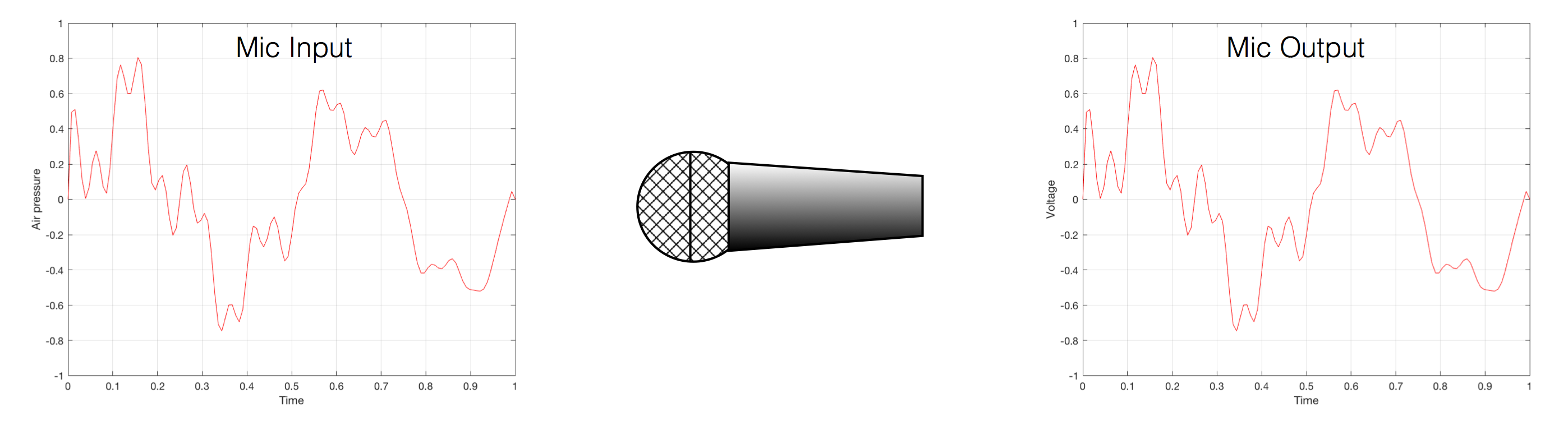Fig 1. Notice that (in theory, and ignoring a lot of things…) the change in air pressure over time at the input of the microphone is identical to the change in voltage over time at its output. Of course, this is not true in real life – microphones lie like a cheap rug…

At this point in the conversation, I’ll make a point to say that, in theory, we could “zoom in” on either of those two curves shown in Figure 1 and see more and more details. This is like looking at a map of Canada – it has lots of crinkly, jagged lines. If you zoom in and look at  the map of Newfoundland and Labrador, you’ll see that it has finer, crinkly, jagged lines. If you zoom in further, and stand where the water meets the shore in Trepassey and take a photo of your feet, you could copy it to draw a map of the line of where the water comes in around the rocks – and your toes – and you would wind up with even finer, crinkly, jagged lines… You could take this even further and get down to a microscopic or molecular level – but you get the idea… The point is that, in theory, both of the plots in Figure 1 have infinite resolution, both in time and in air pressure or voltage.

Now, let’s say that you wanted to take that microphone’s output and transmit it through a bunch of devices and wires that, in theory, all do nothing to the signal. Let’s say, for example, that you take the mic’s output, send it through a wire to a box that makes the signal twice as loud. Then take the output of that box and send it through a wire to another box that makes it half as loud. You take the output of that box and send it through a wire to a measuring device. What will you see? Unfortunately, none of the wires or boxes in the chain can be perfect, so you’ll probably see the signal plus something else which we’ll call the “error” in the system’s output. We can call it the error because, if we measure the input voltage and the output voltage at any one instant, we’ll probably see that they’re not identical. Since they should be identical, then the system must be making a mistake in transmitting the signal – so it makes errors…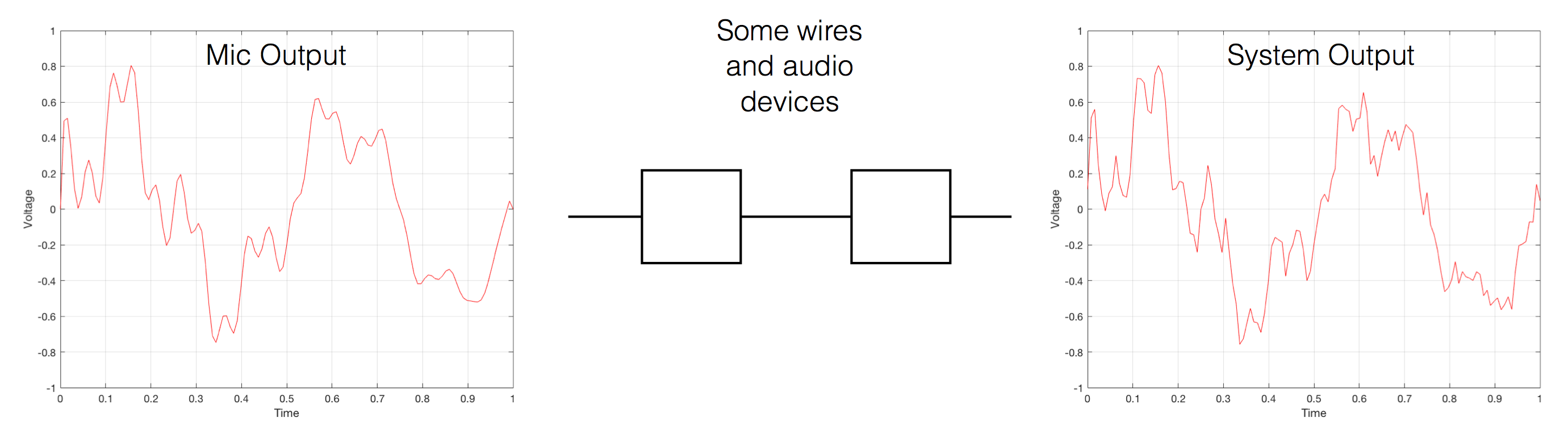Fig 2. If you send an audio signal through some wires and devices that (in theory) do nothing to the signal, you’ll find out that they add some extra stuff that you don’t want.

Pedantic Sidebar: Some people will call that error that the system adds to the signal “noise” – but I’m not going to call it that. This is because “noise” is a specific thing – noise is random – so if it’s not random, it’s not noise. Also, although the signal has been distorted (in that the output of the system is not identical to the input) I won’t call it “distortion” either, since distortion is a name that’s given to something that happens to the signal because the signal is there. (We would probably get at least some of the error out of our system even if we didn’t send any audio into it.) So, we could be slightly geeky and adequately vague and call the extra stuff “Distortion plus noise” but not “THD+N” – which stands for “Total Harmonic Distortion Plus Noise” – because not all kinds of distortion will produce a harmonic of the signal… but I’m getting ahead of myself…

So, we want to transmit (or store) the audio signal – but we want to reduce the noise caused by the transmission (or storage) system. One way to do this is to spend more money on your system. Use wires with better shielding, amplifiers with lower noise floors, bigger power supplies so that you don’t come close to their limits, run your magnetic tape twice as fast, and so on and so on. Or, you could convert the analogue signal (remember that it’s analogous to the change in air pressure over time) to one that is represented (and therefore transmitted or stored) digitally instead.

What does this mean?

## Conversion from analogue to digital and back (but skipping important details)

In the example above, we made a varying voltage that was analogous to the varying air pressure. If we wanted to store this, we could do it by varying the amount of magnetism on a wire or a coating on a tape, for example. Or we could cut a wiggly groove in a bit of vinyl that has a similar shape to the curve in the plots in Figure 1. Or, we could do something else: we could get a metronome (or a clock) and make a measurement of the voltage every time the metronome clicks, and write down the measurements.

For example, let’s zoom in on the first little bit of the signal in the plots in Figure 1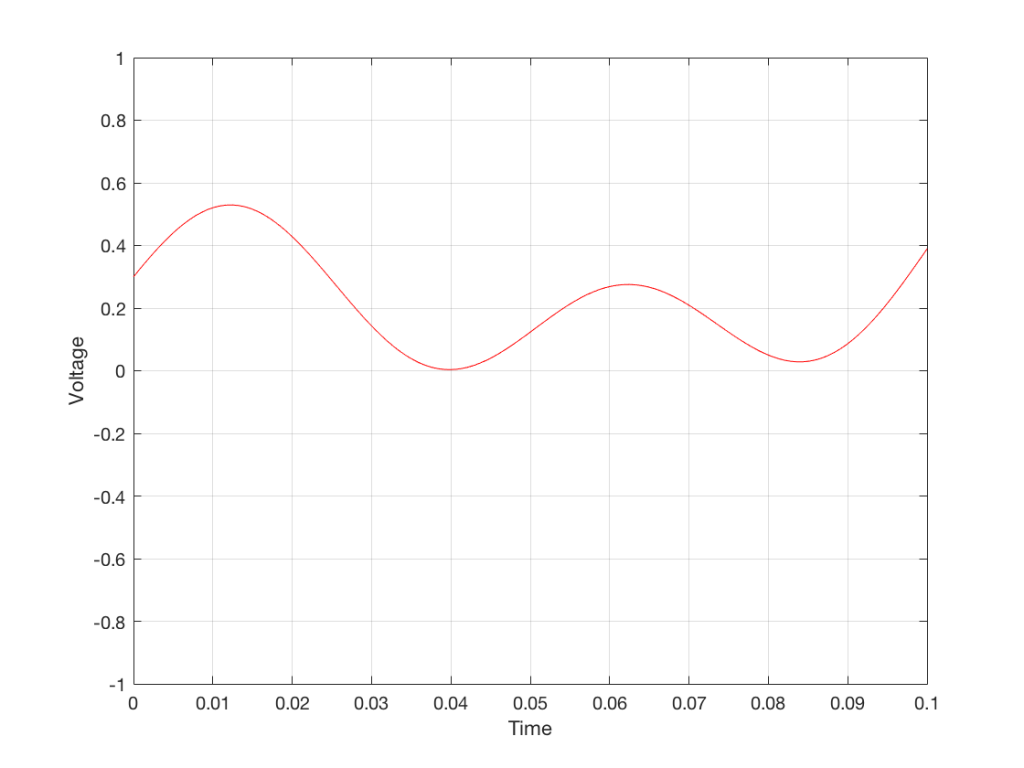Fig. 3 The same curve as was shown in Figure 1 – but zoomed in to the very beginning.

We’ll then put on a metronome and make a measurement of the voltage every time we hear the metronome click…

We can then keep the measurements (remembering how often we made them…) and write them down like this:

0.3000
0.4950
0.5089
0.3351
0.1116
0.0043
0.0678
0.2081
0.2754
0.2042
0.0730
0.0345
0.1775

We can store this series of numbers on a computer’s hard disk, for example. We can then come back tomorrow, and convert the measurements to voltages. First we read the measurements, and create the appropriate voltage…

We then make a “staircase” waveform by “holding” those voltages until the next value comes in.

All we need to do then is to use a low-pass filter to smooth out the hard edges of the staircase.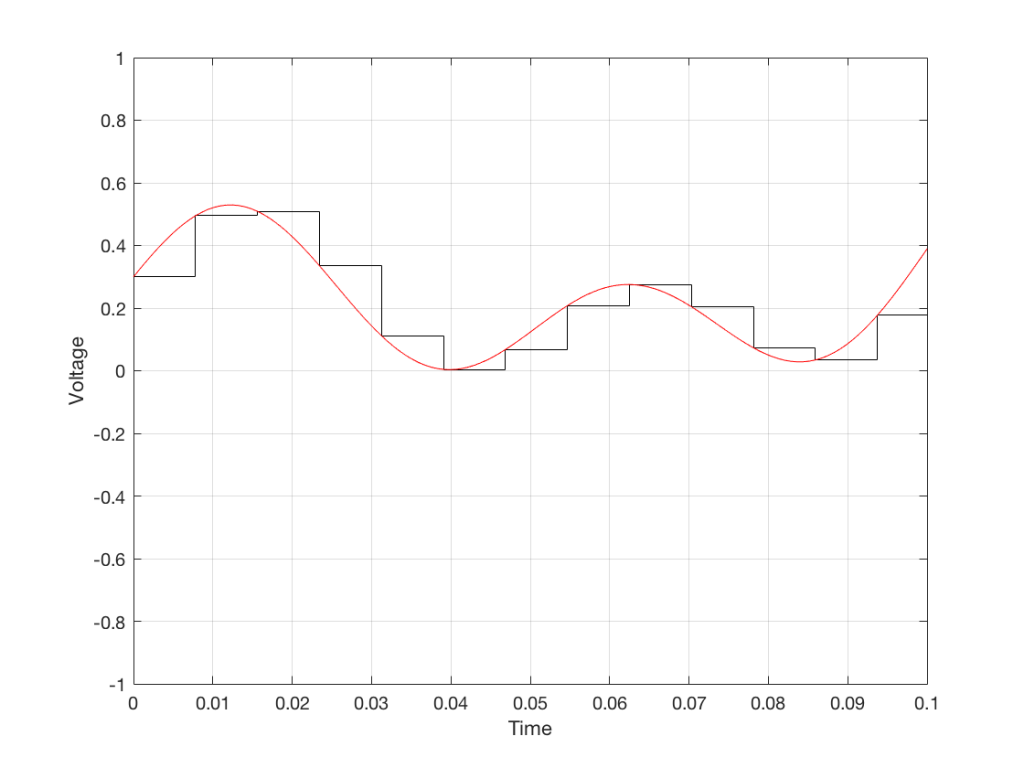Fig 7. When we smooth out the staircase, we get back the original signal (in red).

So, in this example, we’ve gone from an analogue signal (the red curve in Figure 3) to a digital signal (the series of numbers), and back to an analogue signal (the red curve in Figure 7).

In some ways, this is a bit like the way a movie works. When you watch a movie, you see a series of still photographs, probably taken at a rate of 24 pictures (or frames) per second. If you play those photos back at the same rate (24 fps or frames per second), you think you see movement. However, this is because your eyes and brain aren’t fast enough to see 24 individual photos per second – so you are fooled into thinking that things on the screen are moving.

However, digital audio is slightly different from film in two ways:

• The sound (equivalent to the movement in the film) is actually happening. It’s not a trick that relies on your ears and brain being too slow.
• If, when you were filming the movie, something were to happen between frames (say, the flash of a gunshot, for example) then it would never be caught on film. This is because the photos are discrete moments in time – and what happens between them is lost. However, if something were to make a very, very short sound between two samples (two measurements) in the digital audio signal – it would not be lost. This is because of something that happens at the beginning of the chain that I haven’t described… yet…

However, there are some “artefacts” (a fancy term for “weird errors”) that are present both in film and in digital audio that we should talk about.

The first is an error that happens when you mess around with the rate at which you take the measurements (called the “sampling rate”) or the photos (called the “frame rate”) – and, more importantly, when you need to worry about this. Let’s say that you make a film at 24 fps. If you play this back at a higher frame rate, then things will move very quickly (like old-fashioned baseball movies…). If you play them back at a lower frame rate, then things move in slow motion. So, for things to look “normal” you have to play the movie at the same rate that it was filmed. However, as longs no one is looking, you can transfer the movie as fast as you like. For example, if you wanted to copy the film, you could set up a movie camera so it was pointing at a movie screen and film the film. As long as the movie on the screen is running in sync with the camera, you can do this at any frame rate you like. But you’ll have to watch the copy at the same frame rate as the original film…

The second is an easy artefact to recognise. If you see a car accelerating from 0 to something fast on film, you’ll see the wheels of the car start to get faster and faster, then, as the car gets faster, the wheels slow down, stop, and then start going backwards… This does not happen in real life (unless you’re in a place lit with flashing lights like fluorescent bulbs or LED’s). I’ll do a posting explaining why this happens – but the thing to remember here is that the speed of the wheel rotation that you see on the film (the one that’s actually captured by the filming…) is not the real rotational speed of the wheel. However, those two rotational speeds are related to each other (and to the frame rate of the film). If you change the real rotational rate or the frame rate, you’ll change the rotational rate in the film. So, we call this effect “aliasing” because it’s a false version (an alias) of the real thing – but it’s always the same alias (assuming you repeat the conditions…) Digital audio can also suffer from aliasing, but in this case, you put in one frequency (which is actually the same as a rotational speed) and you get out another one. This is not the same as harmonic distortion, since the frequency that you get out is due to a relationship between the original frequency and the sampling rate, so the result is almost never a multiple of the input frequency.

## Some details that I left out…

One of the things I said above was something like “we measure the voltage and store the results” and the example I gave was a nice series of numbers that only had 4 digits after the decimal point. This statement has some implications that we need to discuss.

Let’s say that I have a thing that I need to measure. For example, Figure 8 shows a piece of metal, and I want to measure its width.

Using my ruler, I can see that this piece of metal is about 57 mm wide. However, if I were geeky (and I am) I would say that this is not precise enough – and therefore it’s not accurate. The problem is that my ruler is only graduated in millimetres. So, if I try to measure anything that is not exactly an integer number of mm long, I’ll either have to guess (and be wrong) or round the measurement to the nearest millimetre (and be wrong).

So, if I wanted you to make a piece of metal the same width as my piece of metal, and I used the ruler in Figure 8, we would probably wind up with metal pieces of two different widths. In order to make this better, we need a better ruler – like the one in Figure 9.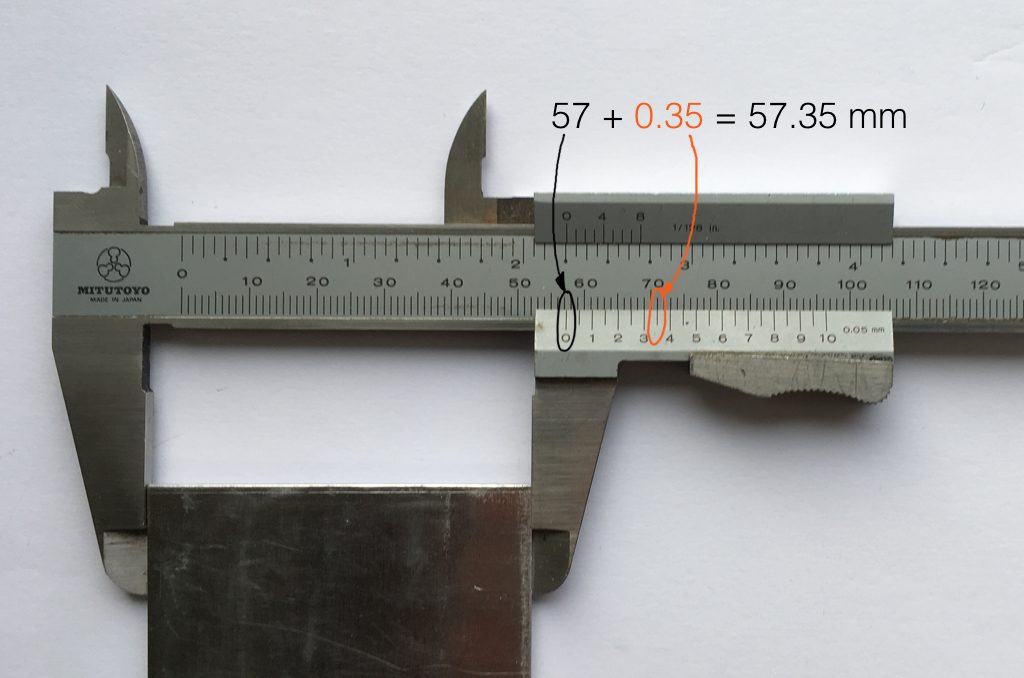Fig 9. The same piece of metal being measured with a vernier caliper. This gives us additional precision (down to 0.05 mm) so we can make a more accurate measurement.

Figure 9 shows a vernier caliper (a fancy type of ruler) being used to measure the same piece of metal. The caliper has a resolution of 0.05 mm instead of the 1 mm available on the ruler in Figure 8. So, we can make a much more accurate measurement of the metal because we have a measuring device with a higher precision.

The conversion of a digital audio signal is the same. As I said above, we measure the voltage of the electrical signal, and transmit (or store) the measurement. The question is: how accurate and precise is your measurement? As we saw above, this is (partly) determined by how many digits are in the number that you use when you “write down” the measurement.

Since the voltage measurements in digital audio are recorded in binary rather than decimal (we use 0 and 1 to write down the number instead of 0 up to 9) then we use Binary digITS – or “bits” instead of decimal digits (which are not called “dits”). The number of bits we have in the number that we write down (partly) determines the precision of the measurement of the voltage – and therefore (possibly), our accuracy…

Just like the example of the ruler in Figure 8, above, we have a limited resolution in our measurement. For example, if we had only 4 bits to work with then the waveform in 4 – the one we have to measure – would be measured with the “ruler” shown on the left side of Figure 10, below.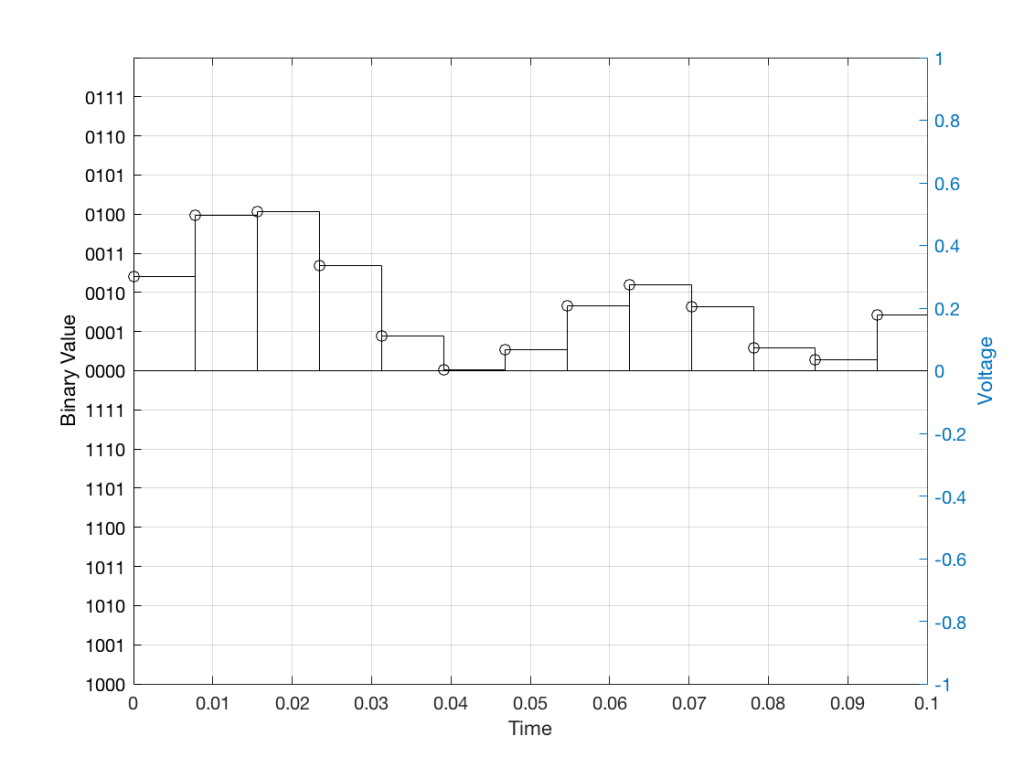Fig 10: The waveform from Figure 4 as a voltage (notice the Y-axis on the right). We have to measure these values using the ruler with the resolution shown on the Y-axis on the left.

When we do this, we have to round off the value to the nearest “tick” on our ruler, as shown in Figure 11.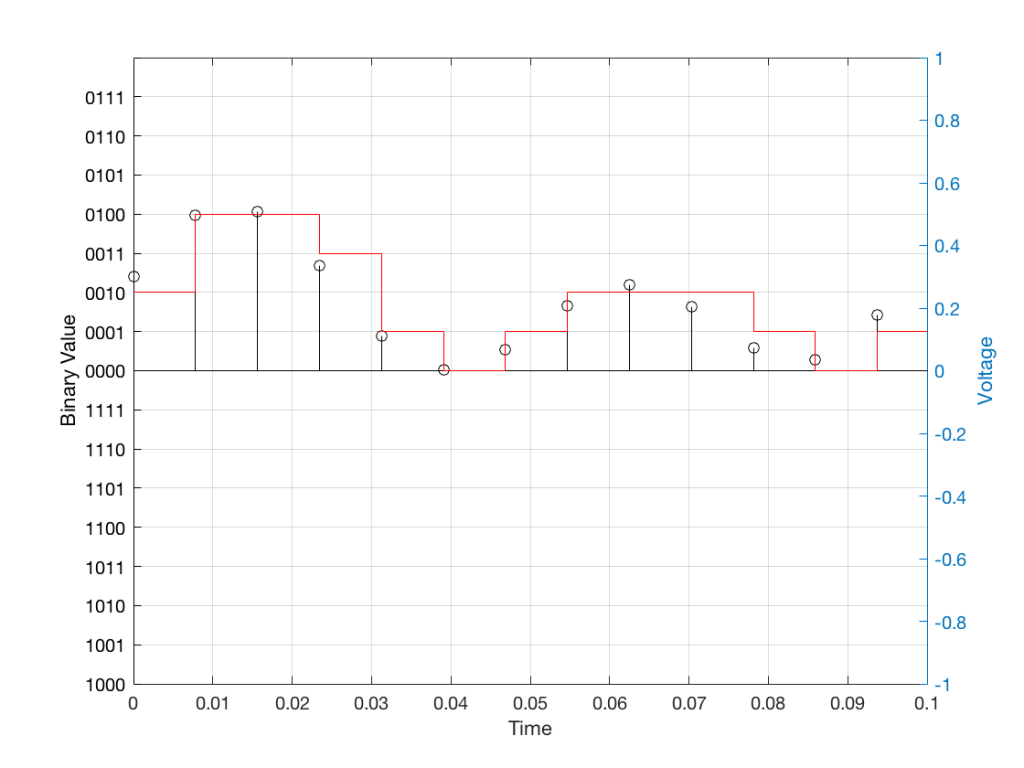Fig 11: The values from figure 10 (shown as the circles) rounded off to the nearest value on our 4-bit ruler (the red staircase).

Using this “ruler” which gives a write-down-able “quantity” to the measurement, we get the following values for the red staircase:

0010
0100
0100
0011
0001
0000
0001
0010
0010
0010
0001
0000
0001

When we “play these back” we get the staircase again, shown in Figure 12.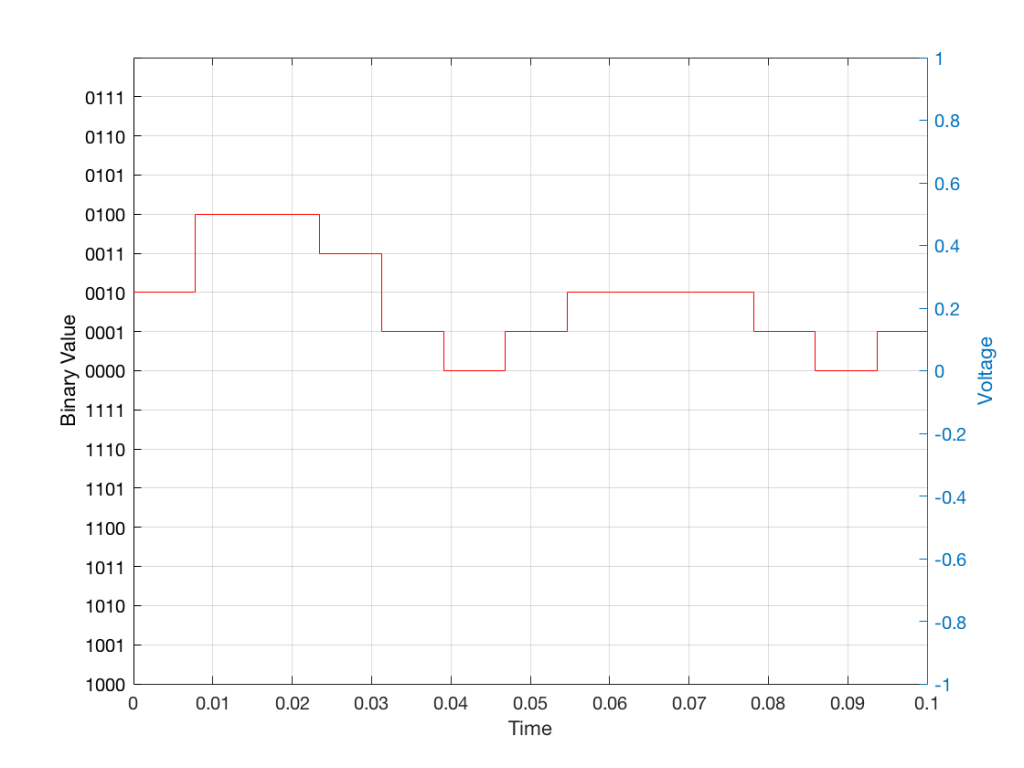Fig 12: The output of the measurements. Notice that all values sit exactly on one of the values for the “ruler” on the left Y-axis of the plot.

Of course, this means that, by rounding off the values, we have introduced an error in the system (just like the measurement in Figure 8 has a bigger error than the one in Figure 9). We can calculate this error if we just subtract the original signal from the output signal (in other words, Figure 12 minus Figure 10) to get Figure 13.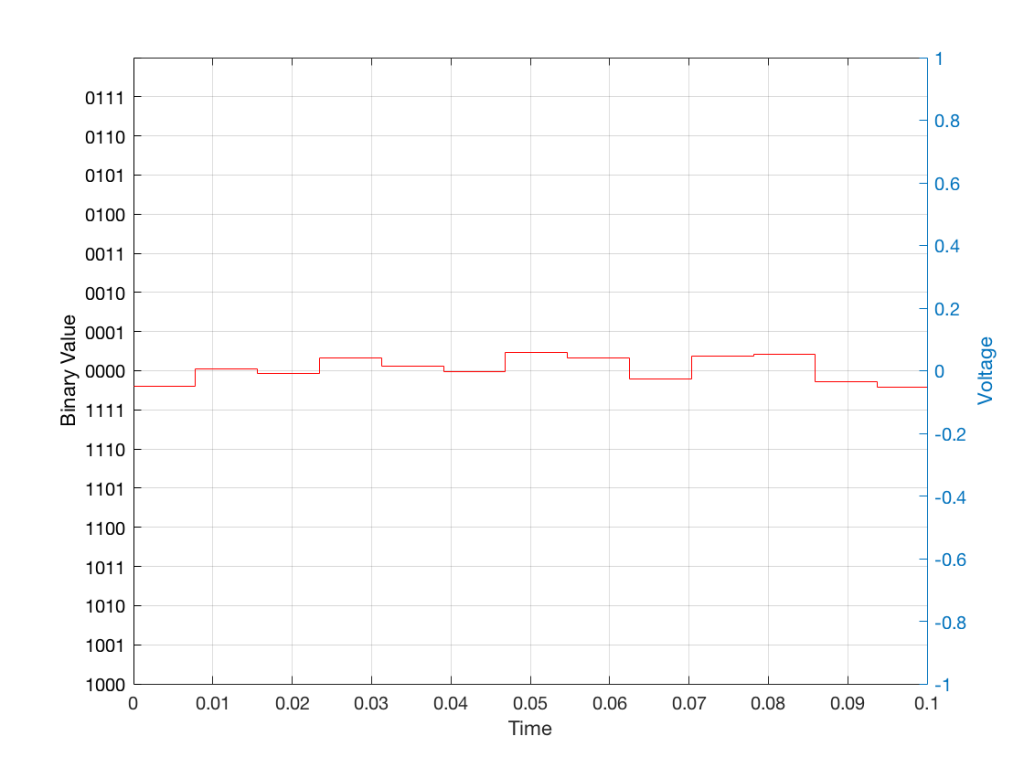Fig 13: The error that we produced due to the rounding off of the signal when we did the measurements. Notice that the error is always less than 0.5 of a “tick” of the ruler on the left Y-axis.

In order to improve our accuracy of the measurement, we have to increase the precision of the values. We can do this by adding an extra digit (or bit) to the number that we use to record the value.

If we were using decimal numbers (0-9) then adding an extra digit to the number would give us 10 times as many possibilities. (For example, if we were using 4 digits after the decimal in the example at the start of this posting, we have a total of 10,000 possible values – 0.0000 to 0.9999. If we add one more digit, we increase the resolution to 100,000 possible values – 0.00000 to 0.99999 ).

In binary, adding one extra digit gives us twice as many “ticks” on the ruler. So, using 4 bits gives us 16 possible values. Increasing to 5 bits gives us 32 possible values.

If you’re listening to a CD, then the individual measurements of each voltage – the “sample values” – are stored with 16 bits, which means that we have 65,536 possible values to pick from.

Remember that this means that we have more “ticks” on our ruler – but we don’t necessarily increase its range. So, for example, we’re still measuring a voltage from -1 V to 1 V – we just have more and more resolution to do that measurement with.

## Error #1

Finally! We get to the beginning of the point of the posting in the first place. My whole reason for starting this series of postings was to talk about errors in digital audio.

So, the first one to talk about is whether we have “bit matching” in a system where we expect to do so. For example, if you look at the S/P-DIF output of a good-old-fashioned CD player, do the sample values that are transmitted on that wire identical to the ones on the disc?

This is a fairly easy test to make (in theory). All you have to do is to record the digital signal on the S/P-DIF output of your CD player, subtract the original signal that’s on the disc (making sure that you have done your time alignment correctly). If you have anything other than nothing left over, then something went wrong somewhere.

If the result of this test is that you do NOT get nothing remaining, you cannot jump in head first and say that your S/P-DIF output is not working properly. For example, some sound cards have a sampling rate converter at their digital input. So, if you are capturing the CD player’s output using such a sound card on your computer, then perhaps the errors that you see are being produced by your sound card – and not your player.

### A little associated story

This was a method that I used to do the final testing of Wireless Power Link for B&O. I created a little software application that made a signal and sent it out digitally to a Wireless Power Link transmitter (which was running with a resolution of 24 bits – giving us 16,777,216 possible values). I then connected a Wireless Power Link receiver’s output to the same computer. The computer knew how much time it took the signal to get from its output, through the wireless transmission system, back to its input (about 5 ms). So, I took the “output” signal, delayed it by that amount, and then subtracted it from the “input” signal. I then made a detector that counted every bit (instead of every sample) that was incorrect.

The reason I was counting bit errors instead of sample errors was that we wanted to be able to diagnose problems if we found them. If you find out that “this sample is wrong” – you don’t necessarily know whether it was one or more bit errors that caused the problem. By counting bit errors, you have a little more information that can help you diagnose the source of problems when you find them.

Sidebar: since this test was running at 48 kHz and 24 bits with a 2-channel system, that means that there were 2,304,000 bits per second being checked every second

This test ran 24-hours a day continuously for over 11 days. In that time, we found 0 bit errors. That means that we got 0 errors in more than 2,189,721,600,000 bits, which was good.

Now, just before anyone gets excited: that test was run to find out whether the WPL system was able to deliver a bit-perfect output in the absence of any external disturbances. So, the transmitter and the receiver were not moved at any time during the test, and nothing was moved between them – and the result was that the system behaved perfectly.

# B&O Tech: Signal Mixing and Decomposition

#72 in a series of articles about the technology behind Bang & Olufsen loudspeakers

After a previous posting, someone posted this as part of a comment:

“What I’ve been asking myself for a long time: how does a single driver manage to produce two or more frequencies (or a frequency range) at the exact same time? For example a singer singing while the guitar plays in the background. Could you try to explain how this works?”

So, this posting is an attempt to answer that question.

Sound is a change of air pressure over time. That pressure is modulating on top of the day’s average barometric pressure – which is just a measurement of how closely the air particles around you are squeezed together. On a high-pressure day, the air is more densely packed – on a low pressure day, the air is less dense.

When you make a sound, you make slight variations in that pressure – so, for example, when a woofer moves out of a loudspeaker enclosure (a fancy name for “box”) then it pushes the air particles in front of it, and they’re squeezed together, resulting in a compression wave that radiates away from the loudspeaker. When the woofer pulls into the enclosure, it pulls the air particles apart, and you get a rarefaction wave instead. (You can see an animation of this at this posting.)

Let’s make a graph that shows a plot of the acoustic pressure changing over time. This is shown below in Figure 1. When this plot shows a positive number, it means that the air particles are being compressed more than normal. When it’s negative, then they’re being separated more than normal. Without getting into too many details, let’s just say that this is a low frequency. (If you want to get picky, then you’ll see that this is one cycle of a wave that takes 100 ms. Since there are 1000 ms in a second, then this must be a 10 Hertz signal, because 1000 / 100 = 10. That makes it a VERY low frequency by normal audio standards… )

Let’s also look at an example of a higher frequency, shown in Figure 2, below.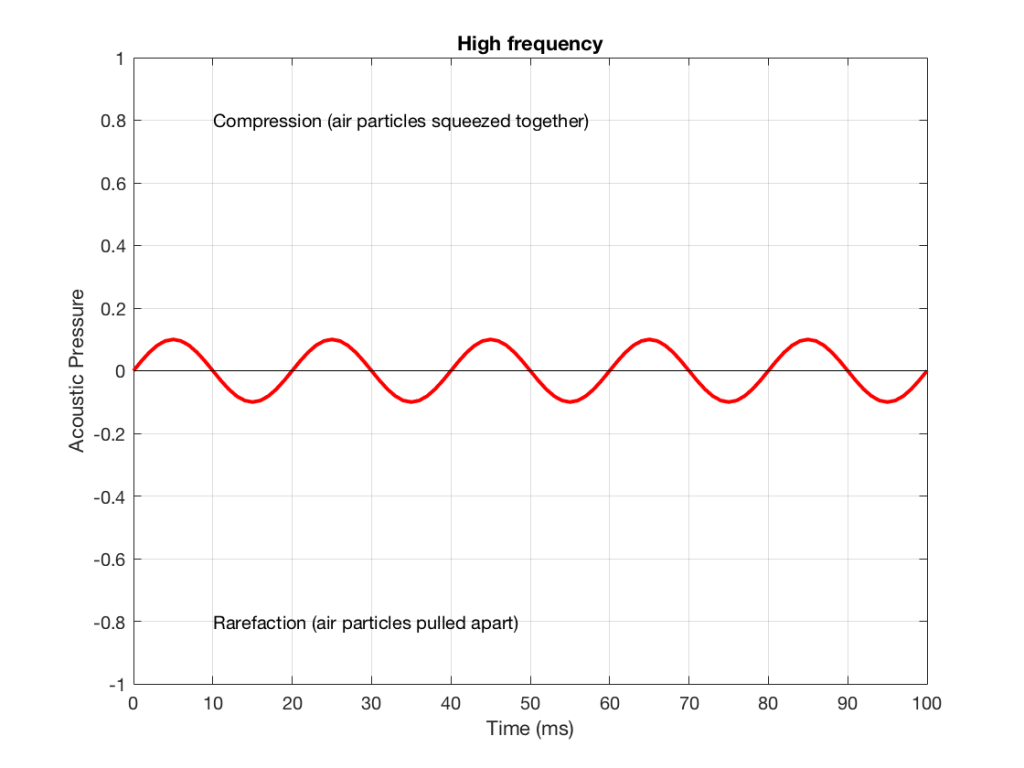Figure 2: A signal with a higher frequency and a lower amplitude than the one shown in Figure 1.

We can see that Figure 2 has a higher frequency signal, because it moves up and down more frequently. It has 5 cycles (5 ‘ups’ and ‘downs’) in the same amount of time that it took the wave in Figure 1 to have 1 cycle – therefore it is 5 times the frequency (and therefore, if you’re being picky, 50 Hz – which, by audio standards, is also a very low frequency, but this is just an example…)

Ignoring that ACTUAL frequencies that are plotted there, let’s pretend for a moment that the low frequency (Figure 1) came from a bass guitar and the higher frequency (Figure 2) came from a singer. If we took those two signals and put them into a mixing console, what does the result look like?

Well, we take the instantaneous value of the signal at one moment in time and add it to the instantaneous value of the other signal at the same time. Let’s do that.

Figure 3 shows the same signal as in Figure 1, but I’ve pointed out the values at two moments in time – at 25 ms and at 50 ms. So, for example, you can see there that, at 25 ms, the value is 0.5 – whatever that means…

Figure 4 shows the same signal as in Figure 2, but I’ve pointed out the values at two same moments in time – at 25 ms and at 50 ms. So, for example, you can see there that, at 25 ms, the value is 0.1 – whatever that means.

We take the value at 25 ms from each of the two signals (0.5 and 0.1) and add them together to get 0.6. This is the value of the signal at the output of the mixer at 25 ms. At 50 ms, the mixer’s output will have a value of 0 (because 0+0 = 0). This is shown graphically below for all of the values of both plots from 0 ms to 100 ms.

So, you can see in Figure 5 what the result will be. This signal contains both the low frequency, shown in Figure 1 and the higher frequency shown in Figure 2. If we send this combined signal to a loudspeaker, then both signals will get reproduced.

One interesting thing to note is that this mixing can also be done in the air. If a bass guitar and a singer are performing a song together, live, then the bass is pushing and pulling the molecules at the same time that the singer’s voice does. So, if at 25 ms, the bass pushes the molecules with a value of 0.5 (whatever that means) and the singer pushes the molecules with a value of 0.1, then your eardrum will be pushed in with a value of 0.6.  So, the summation of the pressure signals happens in the air, just like it does as voltages or voltage measurements in the mixing console.

## Splitting signals apart

Typically, however, a loudspeaker is comprised of more than one driver – for example, a woofer (for the low frequencies) and a tweeter (for the high frequencies). (Of course, some loudspeakers have more than two drivers, but we’re keeping things simple today…)

So, what we do there is to put the total signal, shown in Figure 5, and send it to two circuits that change how loud things are, depending on their frequency. One circuit is called a “low pass filter” because it allows low frequencies to pass through it unchanged, but it reduces the level of higher frequencies. The other circuit is called a “high pass filter” because it allows the high frequencies to pass through it unchanged, but it reduces the level of the lower frequencies. (we won’t talk about how those circuits do that in this posting…)

We can plot the two characteristics of these two circuits – an example of which is shown in Figure 6.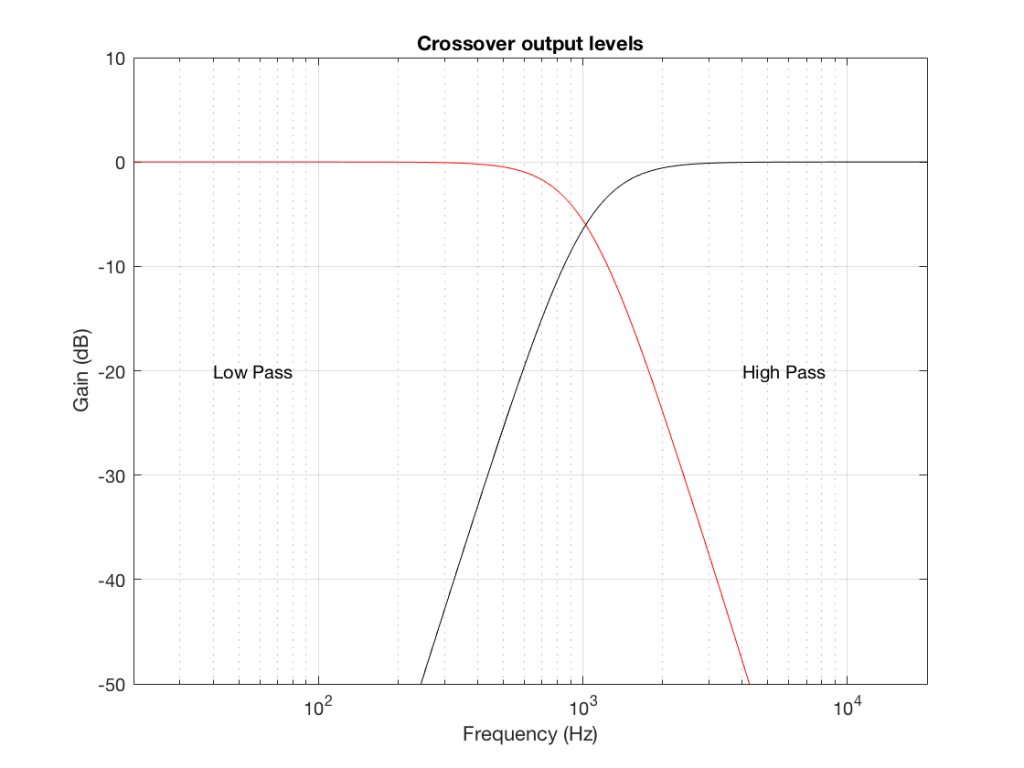Figure 6: An example of the gains applied to a signal by a low pass filter (in red) and a high pass filter (in black). Notice that, at one frequency, both filters have the same output level. That frequency is called the “crossover frequency” because it’s the frequency where the two filters’ responses cross over each other.

IF we send a signal like the one in Figure 5 to a crossover that happens to have a crossover frequency that is between the two frequencies it contains, then the signal will be split into two – one output containing mostly low-frequency components, and the other one containing mostly high-frequency components. Examples of these are shown below.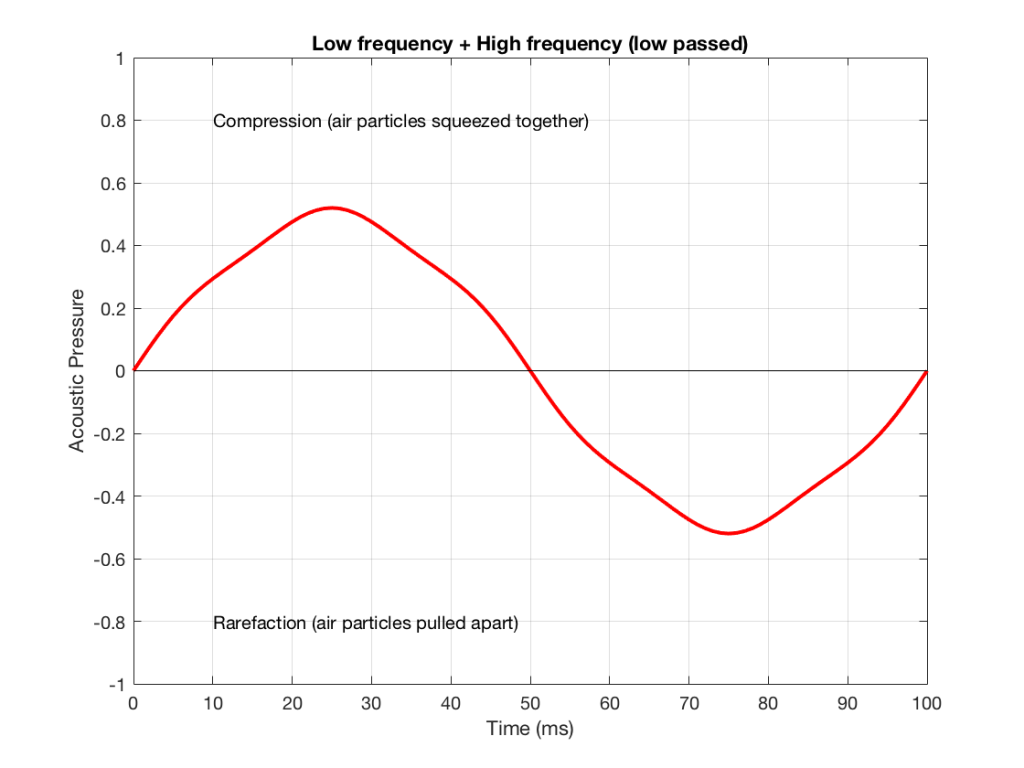Figure 7: An example of the output of the signal from Figure 5, sent though a low-pass filter. Notice that it looks a lot like Figure 1 (the low frequency component of the combined signal) – but there’s a little bit of Figure 2 (the high frequency component) left in there…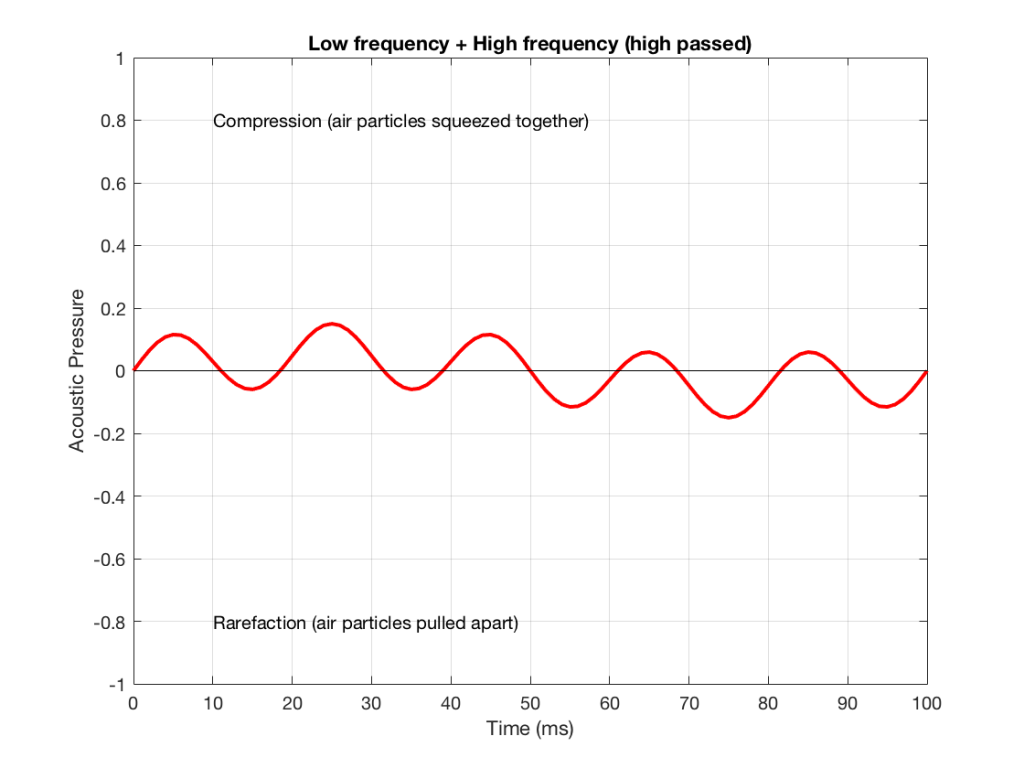Figure 8: An example of the output of the signal from Figure 5, sent though a high-pass filter. Notice that it looks a lot like Figure 2 (the high frequency component of the combined signal) – but there’s a little bit of Figure 1 (the low frequency component) left in there…

NB: Of course, everything I’ve shown here are just examples to make the concept intuitive. The crossover shown in Figure 6 would not work the way I’ve shown it in Figures 7 and 8 because the crossover frequency is too high compared to the 10 Hz and 50 Hz waves that I used in the example. So, please do not make comments talking about how I chose the wrong crossover frequency…

# Probability Density Functions, Part 3

In the last posting, I talked about the effects of a bandpass filter on the probability density function (PDF) of an audio signal. This left the open issue of other filter types. So, below is the continuation of the discussion…

I made noise signals (length 2^16 samples, fs=2^16) with different PDFs, and filtered them as if I were building a three-way loudspeaker with a 4th order Linkwitz-Riley crossover (without including the compensation for the natural responses of the drivers). The crossover frequencies were 200 Hz and 2 kHz (which are just representative, arbitrary values).

So, the filter magnitude responses looked like Figure 1.

The resulting effects on the probability distribution functions are shown below. (Check the last posting for plots of the PDFs of the full-band signals – however note that I made new noise signals, so the magnitude responses won’t match directly.)

The magnitude responses shown in the plots below have been 1/3-octave smoothed – otherwise they look really noisy.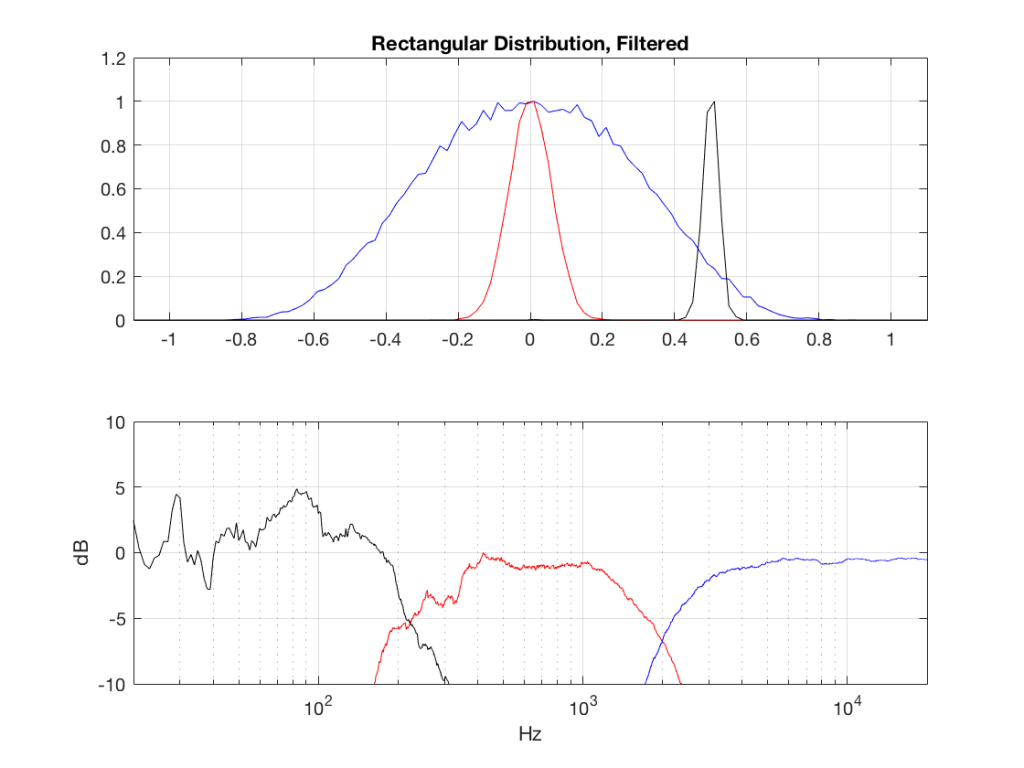Fig 2: PDFs of a noise signal with a rectangular distribution that has been split into the three bands shown in Figure 1. Note the DC offset of the signal, visible in the low-pass output’s PDF.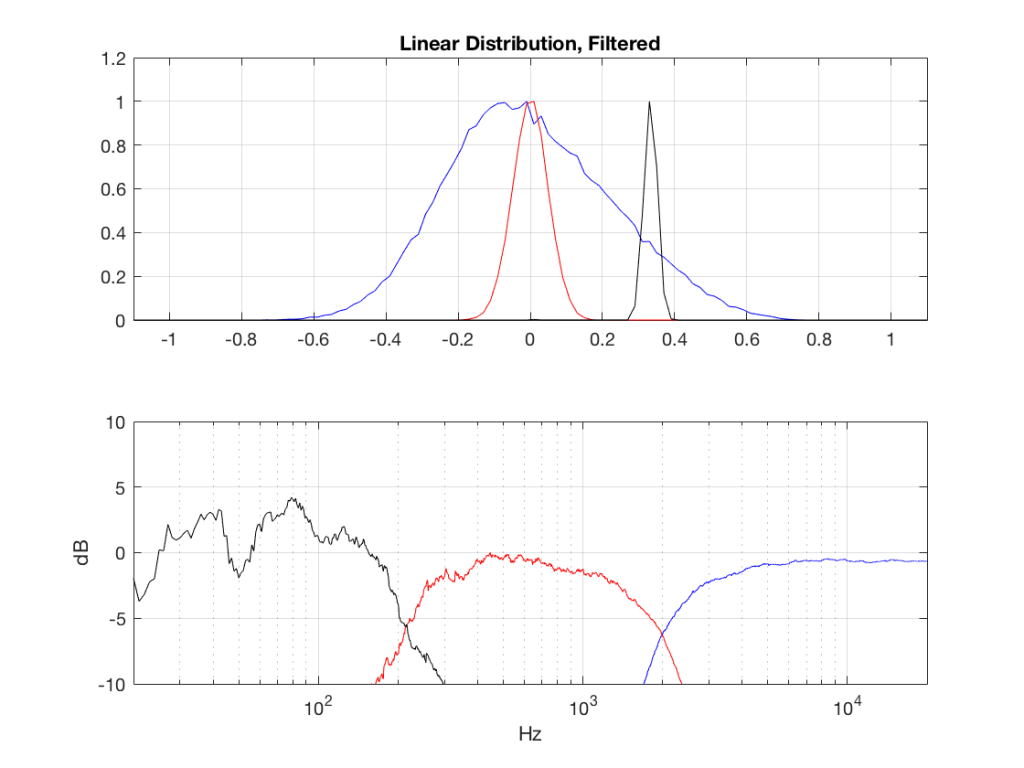Fig 3: PDFs of a noise signal with a linear distribution that has been split into the three bands shown in Figure 1. Note the DC offset of the signal, visible in the low-pass output’s PDF.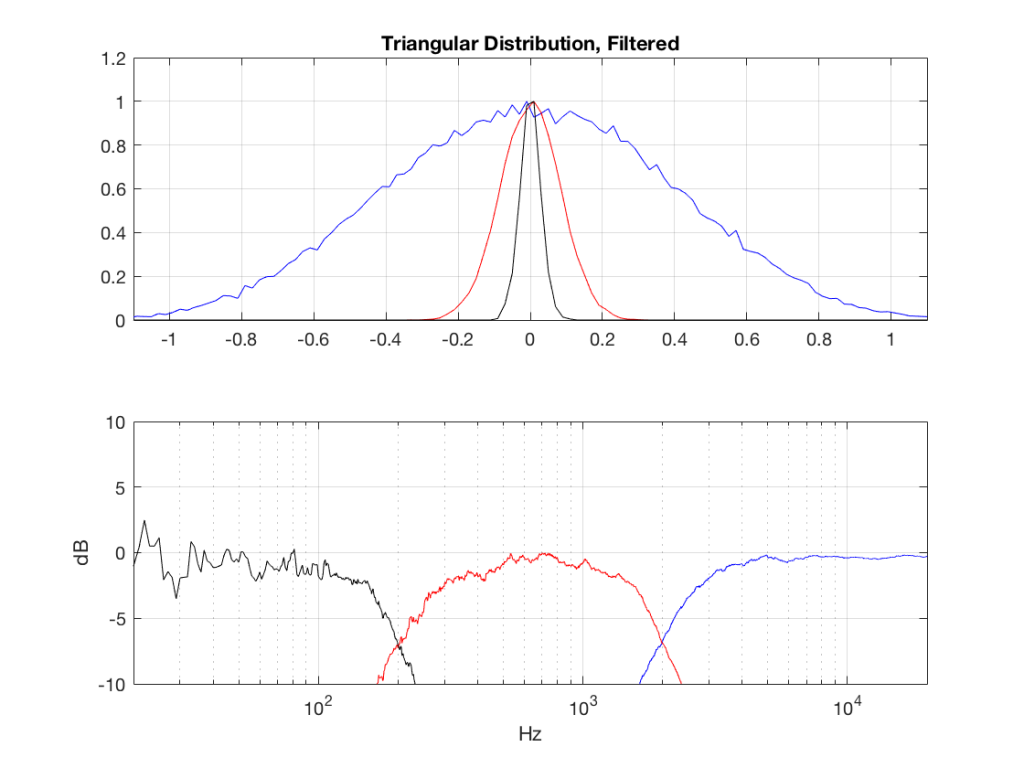Fig 4: PDFs of a noise signal with a triangular distribution that has been split into the three bands shown in Figure 1.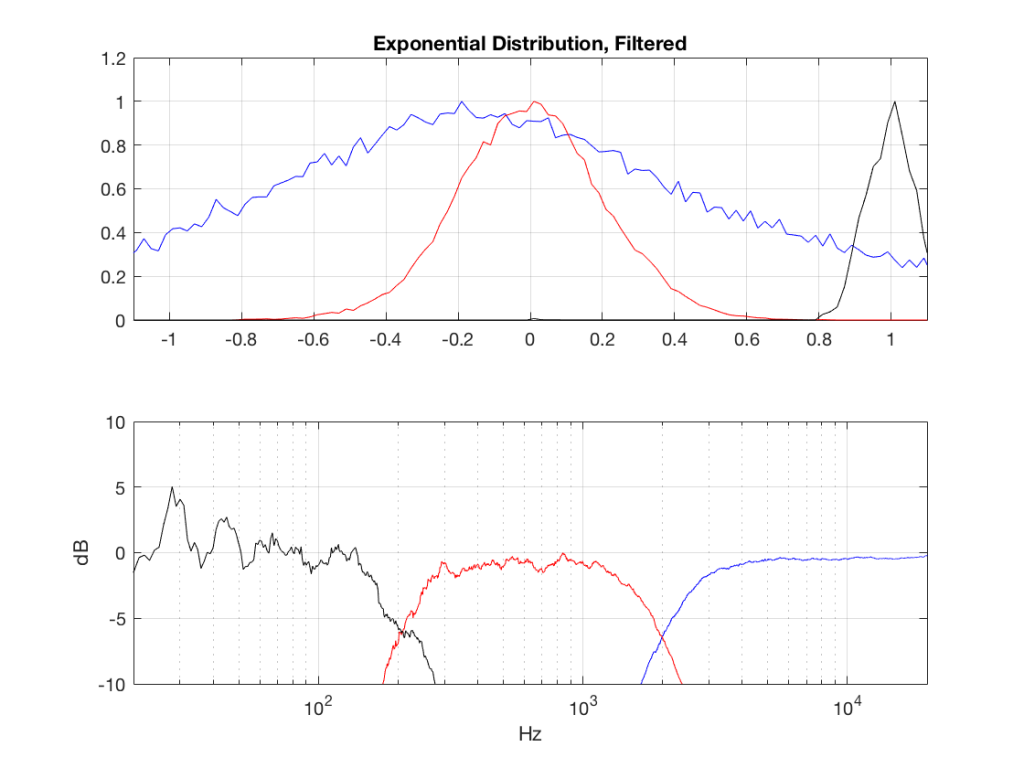Fig 5: PDFs of a noise signal with an exponential distribution that has been split into the three bands shown in Figure 1. Note the DC offset of the signal, visible in the low-pass output’s PDF.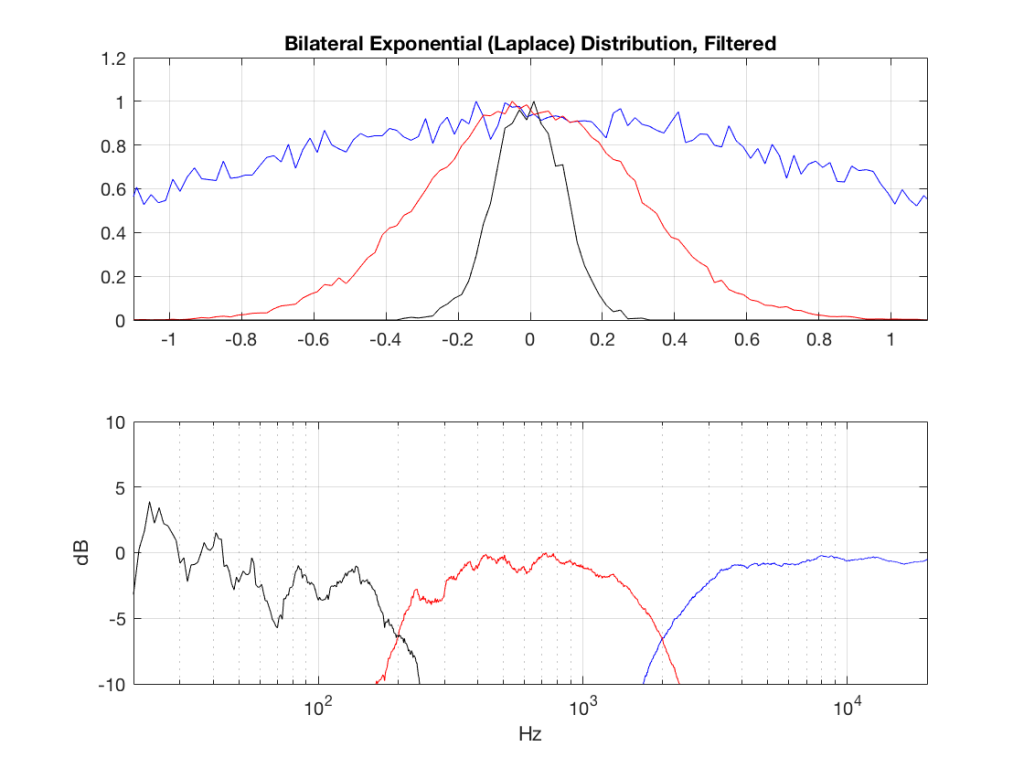Fig 6: PDFs of a noise signal with a Laplacian distribution that has been split into the three bands shown in Figure 1.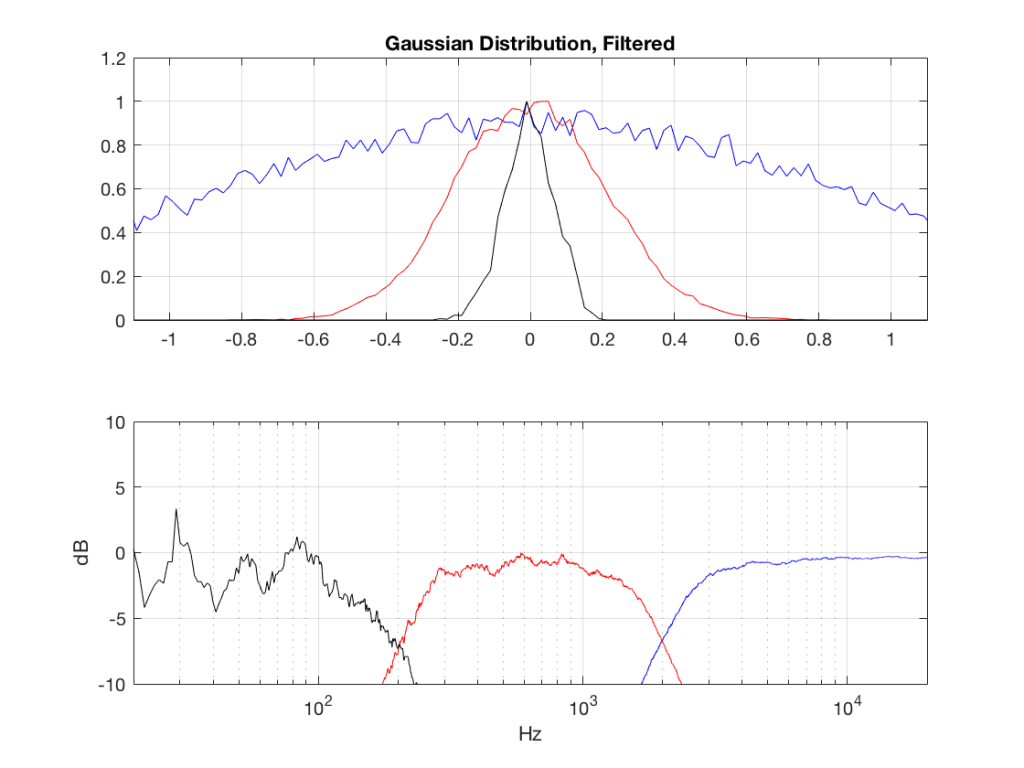Fig 7: PDFs of a noise signal with a Gaussian distribution that has been split into the three bands shown in Figure 1.

## Post-script

This posting has a Part 1 that you’ll find here and a Part 2 that you’ll find here.

# Probability Density Functions, Part 2

In a previous posting, I showed some plots that displayed the probability density functions (or PDF) of a number of commercial audio recordings. (If you are new to the concept of a probability density function, then you might want to at least have a look at that posting before reading further…)

I’ve been doing a little more work on this subject, with some possible implications on how to interpret those plots. Or, perhaps more specifically, with some possible implications on possible conclusions to be drawn from those plots.

# Full-band examples

To start, let’s create some noise with a desired PDF, without imposing any frequency limitations on the signal.

To do this, I’ve ported equations from “Computer Music: Synthesis, Composition, and Performance” by Charles Dodge and Thomas A. Jerse, Schirmer Books, New York (1985) to Matlab. That code is shown below in italics, in case you might want to use it. (No promises are made regarding the code quality… However, I will say that I’ve written the code to be easily understandable, rather than efficient – so don’t make fun of me.) I’ve made the length of the noise samples 2^16 because I like that number. (Actually, it’s for other reasons involving plotting the results of an FFT, and my own laziness regarding frequency scaling – but that’s my business.)

## Uniform (aka Rectangular) Distribution

uniform = rand(2^16, 1);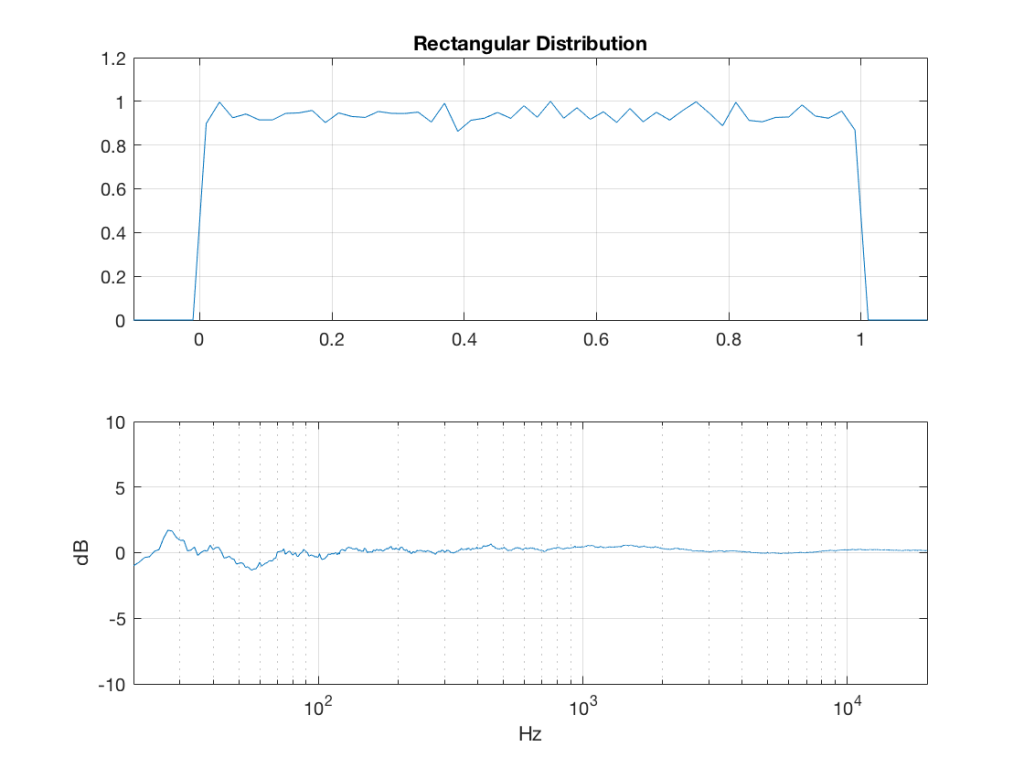Fig 1: The PDF and the spectrum (1-octave smoothed) of a noise signal with a rectangular distribution. Note that there is a DC component, since there are no negative values in the signal.

Of course, as you can see in the plots in Figure 1, the signal is not “perfectly” rectangular, nor is it “perfectly” flat. This is because it’s noise. If I ran exactly the same code again, the result would be different, but also neither perfectly rectangular nor flat. Of course, if I ran the code repeatedly, and averaged the results,  the average would become “better” and “better”.

## Linear Distribution

linear_temp_1 = rand(2^16, 1);

linear_temp_2  = rand(2^16, 1);

temp_indices = find(linear_temp_1 < linear_temp_2);

linear = linear_temp_2;

linear(temp_indices) = linear_temp_1(temp_indices);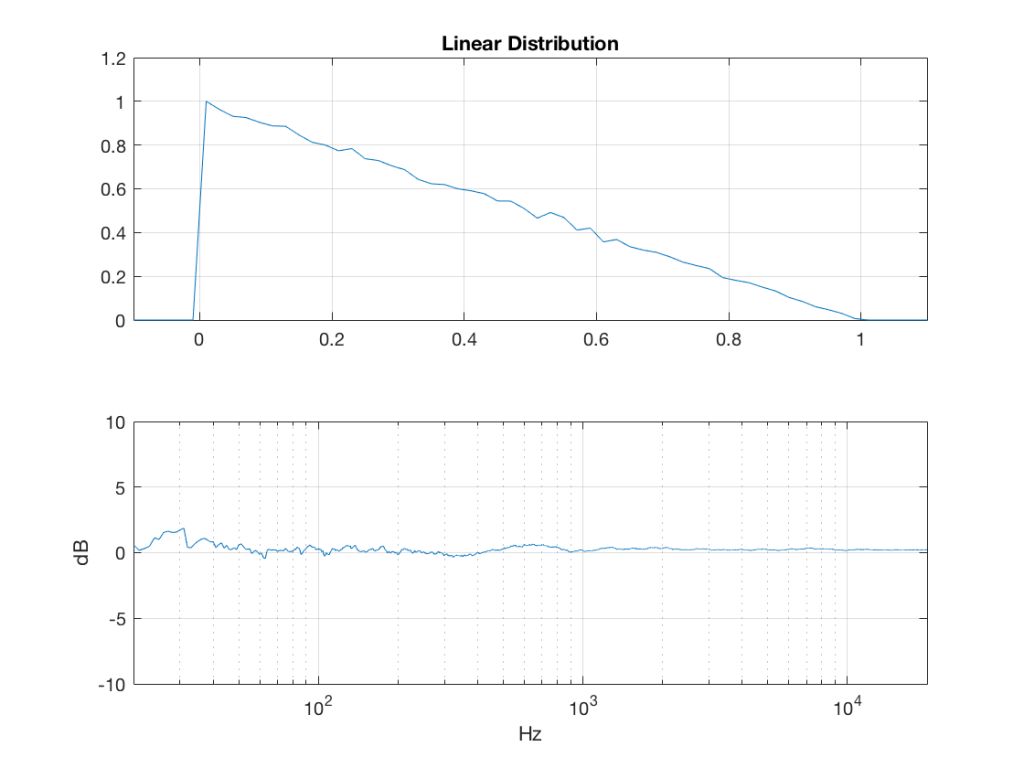Fig 2: The PDF and the spectrum (1-octave smoothed) of a noise signal with a linear distribution. Note that there is a DC component, since there are no negative values in the signal.

## Triangular Distribution

triangular = rand(2^16, 1) – rand(2^16, 1);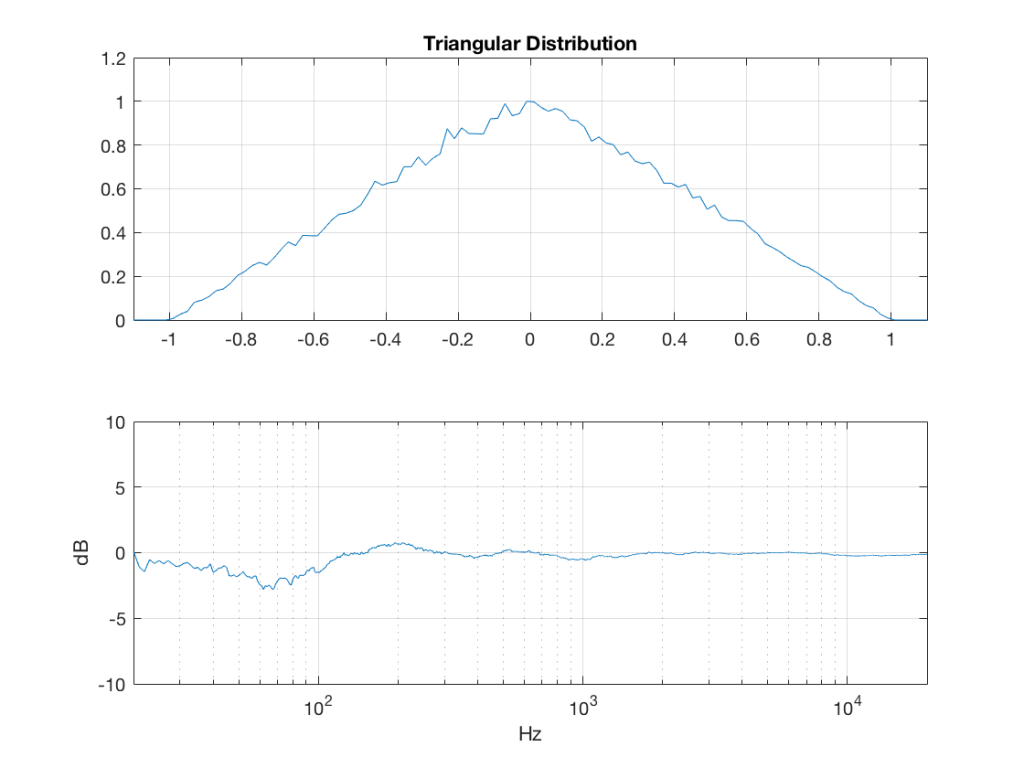Fig 3: The PDF and the spectrum (1-octave smoothed) of a rise signal with a triangular distribution. Note that there is no DC component, since the PDF is symmetrical across the 0 line.

## Exponential Distribution

lambda = 1;     % lambda must be greater than 0

exponential_temp = rand(2^16, 1) / lambda;

if any(exponential_temp == 0)    % ensure that no values of exponential_temp are 0

end

exponential = -log(exponential_temp);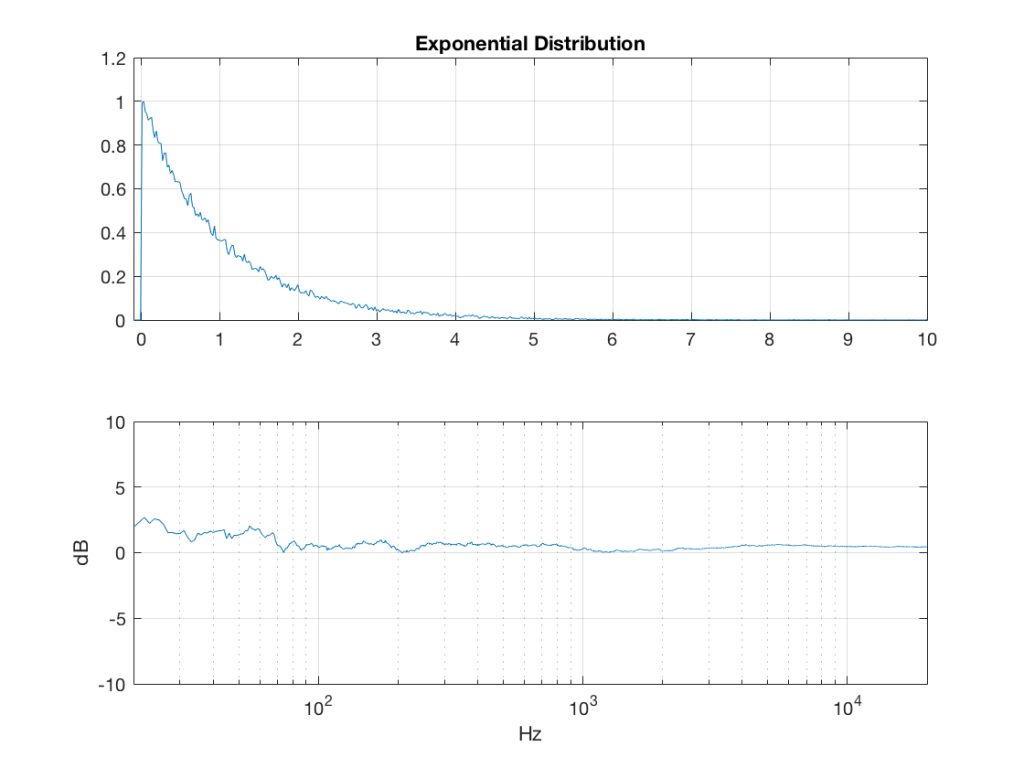Fig 4: The PDF and the spectrum (1-octave smoothed) of a rise signal with an exponential distribution. Note that there is a DC component, since there are no negative values in the signal. Note as well that the values can be significantly higher than 1, so you might incur clipping if you use this without thinking…

## Bilateral Exponential Distribution (aka Laplacian)

lambda = 1;     % must be greater than 0

bilex_temp = 2 * rand(2^16, 1);

% check that no values of bilex_temp are 0 or 2

if any(bilex_temp == 0)

end

bilex_lessthan1 = find(bilex_temp <= 1);

bilex(bilex_lessthan1, 1) = log(bilex_temp(bilex_lessthan1)) / lambda;

bilex_greaterthan1 = find(bilex_temp > 1);

bilex_temp(bilex_greaterthan1) = 2 – bilex_temp(bilex_greaterthan1);

bilex(bilex_greaterthan1, 1) = -log(bilex_temp(bilex_greaterthan1)) / lambda;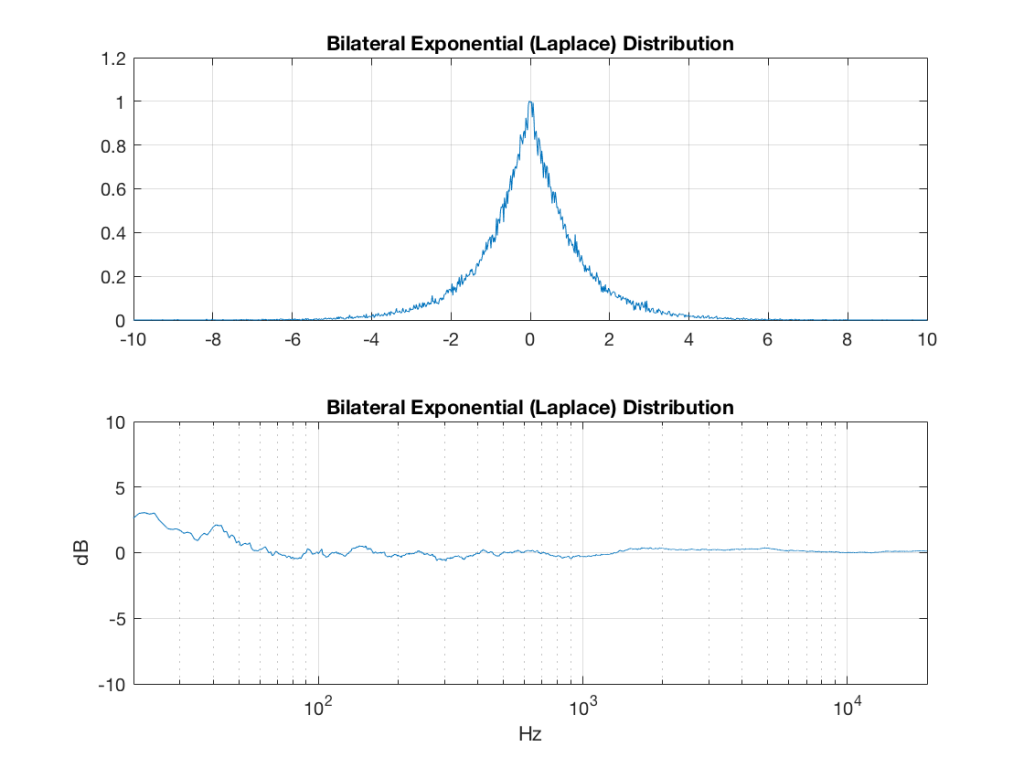Fig 5: The PDF and the spectrum (1-octave smoothed) of a rise signal with a bilateral exponential distribution. Note that there is no DC component, since the PDF is symmetrical across the 0 line. Note as well that the values can be significantly higher than 1 (or less than -1), so you might incur clipping if you use this without thinking…

## Gaussian

sigma = 1;

xmu = 0;   % offset

n = 100; % number of random number vectors used to create final vector (more is better)

xnover = n/2;

sc = 1/sqrt(n/12);

total = sum(rand(2^16, n), 2);

gaussian = sigma * sc * (total – xnover) + xmu;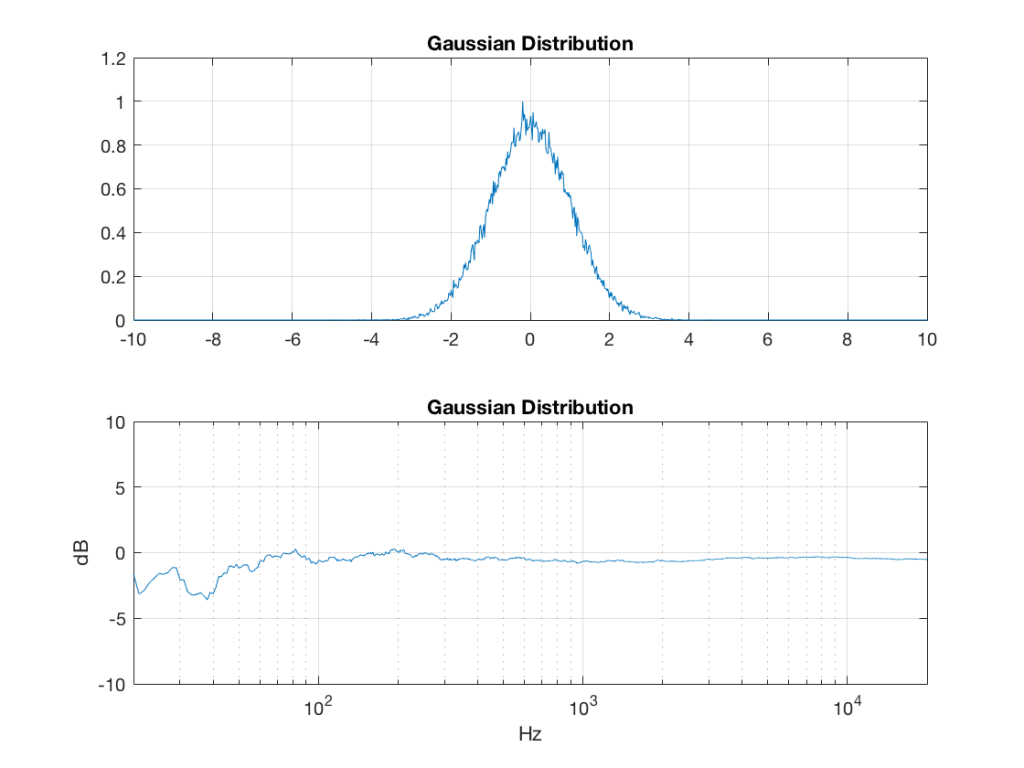Fig 6:  The PDF and the spectrum (1-octave smoothed) of a rise signal with a Gaussian distribution. Note that there is no DC component, since the PDF is symmetrical across the 0 line. Note as well that the values can be significantly higher than 1 (or less than -1), so you might incur clipping if you use this without thinking…

Of course, if you are using Matlab, there is an easier way to get a noise signal with a Gaussian PDF, and that is to use the randn() function.

# The effects of band-passing the signals

What happens to the probability distribution of the signals if we band-limit them? For example, let’s take the signals that were plotted above, and put them through two sets of two second-order Butterworth filters in series, one set producing a high-pass filter at 200 Hz and the other resulting in a low-pass filter at 2 kHz .(This is the same as if we were making a mid-range signal in a 4th-order Linkwitz-Riley crossover, assuming that our midrange drivers had flat magnitude responses far beyond our crossover frequencies, and therefore required no correction in the crossover…)

What happens to our PDF’s as a result of the band limiting? Let’s see…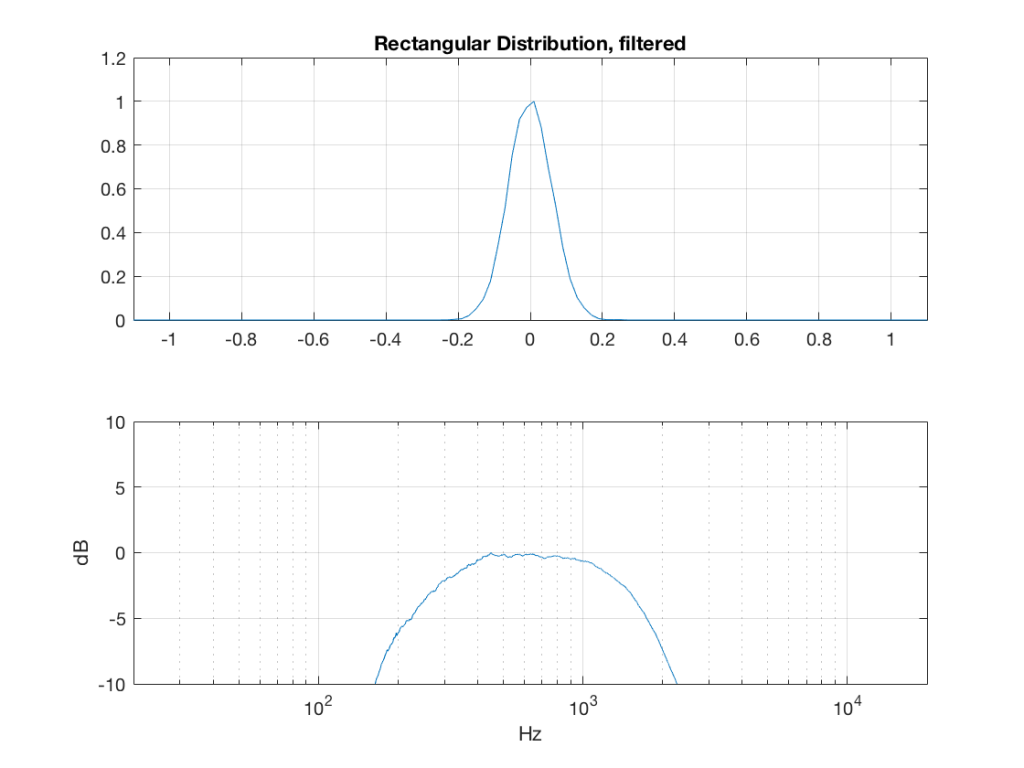Fig 7: The PDF of noise with a rectangular distribution that has been band-limited from 200 Hz to 2 kHz.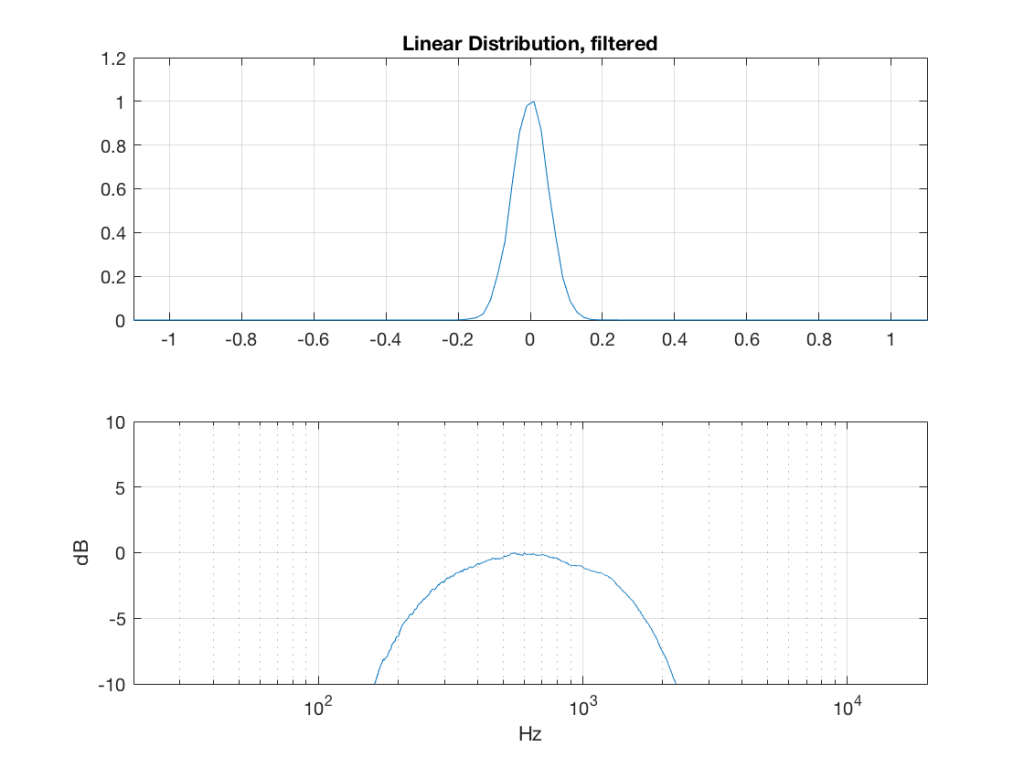Fig 8: The PDF of noise with a linear distribution that has been band-limited from 200 Hz to 2 kHz.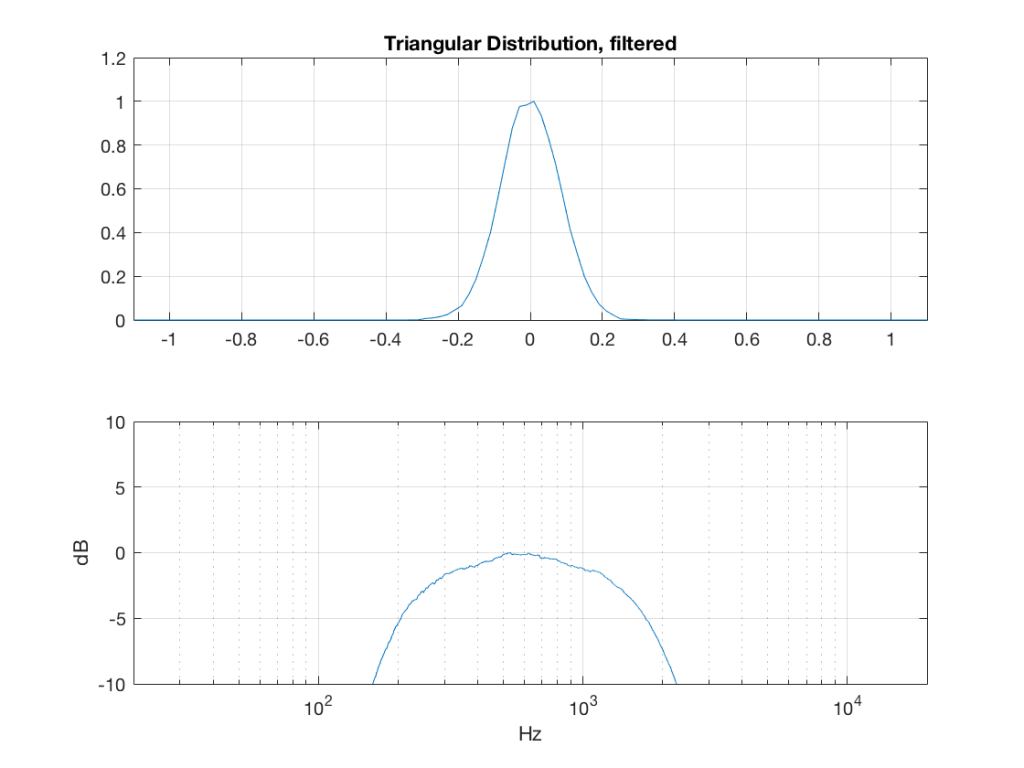Fig 9: The PDF of noise with a triangular distribution that has been band-limited from 200 Hz to 2 kHz.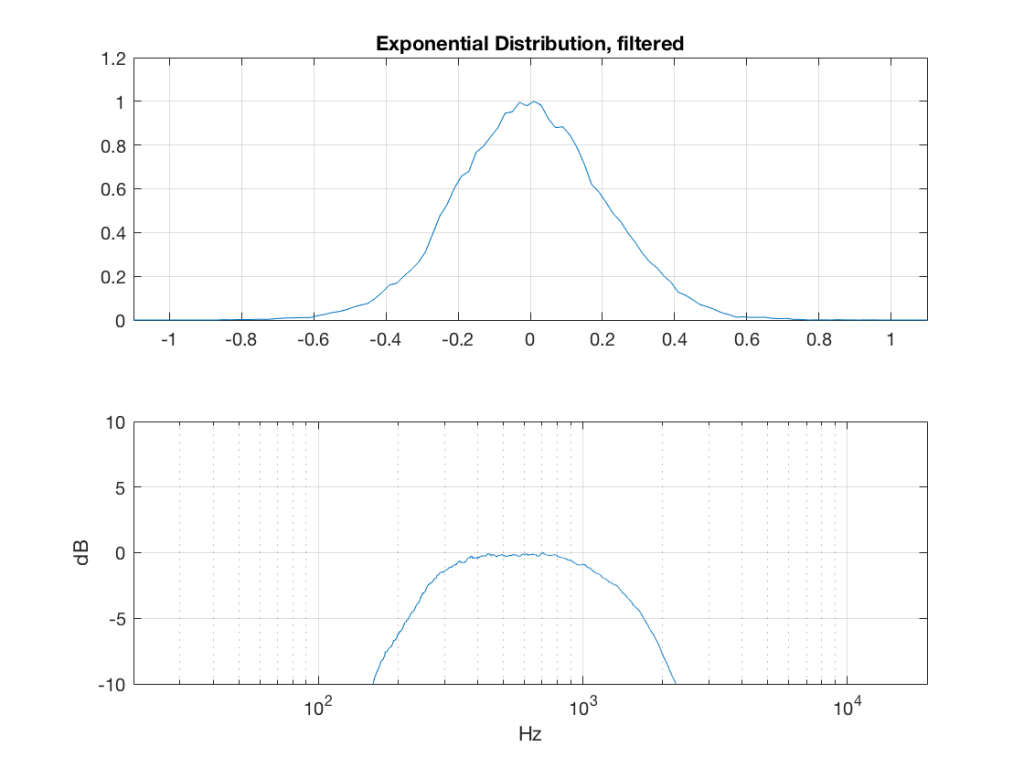Fig 10: The PDF of noise with an exponential distribution that has been band-limited from 200 Hz to 2 kHz.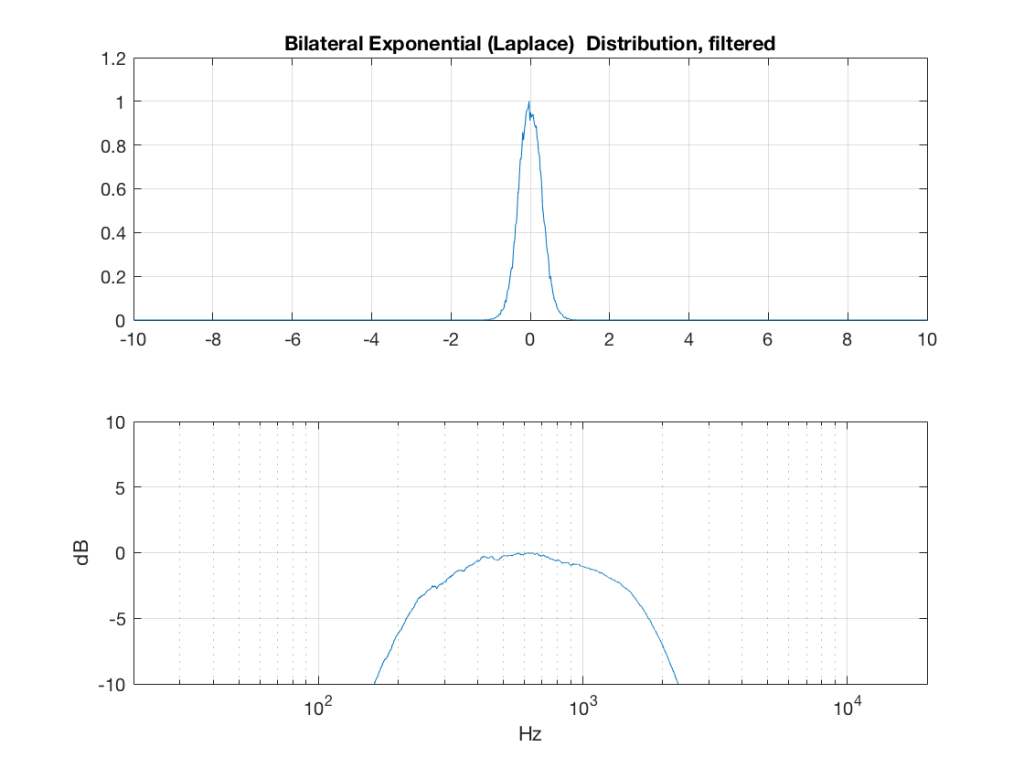Fig 11: The PDF of noise with a Laplacian distribution that has been band-limited from 200 Hz to 2 kHz.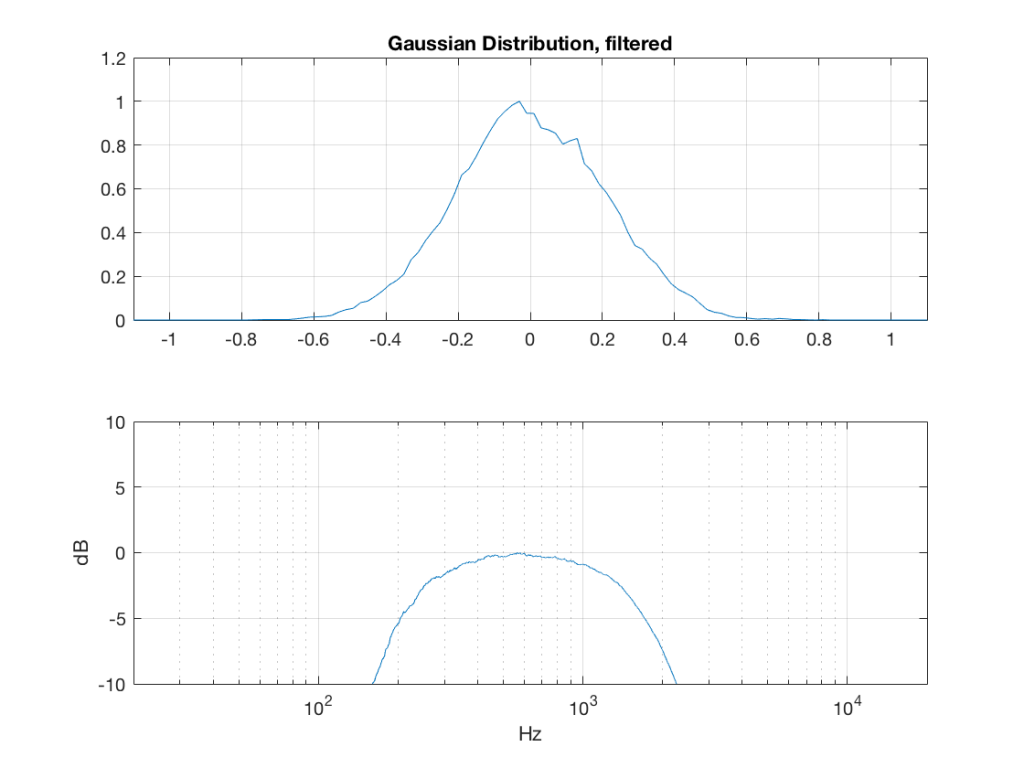Fig 12: The PDF of noise with a Gaussian distribution that has been band-limited from 200 Hz to 2 kHz.

So, what we can see in Figures 7 through 12 (inclusive) is that, regardless of the original PDF of the signal, if you band-limit it, the result has a Gaussian distribution.

And yes, I tried other bandwidths and filter slopes. The result, generally speaking, is the same.

One part of this effect is a little obvious. The high-pass filter (in this case, at 200 Hz) removes the DC component, which makes all of the PDF’s symmetrical around the 0 line.

However, the “punch line” is that, regardless of the distribution of the signal coming into your system (and that can be quite different from song to song as I showed in this posting) the PDF of the signal after band-limiting (say, being sent to your loudspeaker drivers) will be Gaussian-ish.

And, before you ask, “what if you had only put in a high-pass or a low-pass filter?” – that answer is coming in a later posting…

## Post-script

This posting has a Part 1 that you’ll find here, and a Part 3 that you’ll find here.

# 997 Hz?

In my previous posting, I mentioned that I was using a tone at or around 997 Hz to test my signal. In truth, only one of the plots I showed there actually used 997 Hz – but that doesn’t really matter.

The question that I’ll talk about in this posting is “why did I prefer to use 997 Hz instead of 1 kHz as my target frequency?” (I didn’t just randomly choose 997 Hz – it’s a common number that’s often used by people in the audio industry.)

The answer to that question has to do with some considerations on how digital audio equipment and software is tested.

Let’s start by talking a little about how a signal gets a PCM (Pulse-Code Modulation) representation in the digital domain. Note that this is the VERY basic explanation – I’m leaving out a lot of steps here…

We’ll start with a signal like the portion of a sine wave shown in Figure 1.

This signal is continuous – meaning that we can zoom in infinitely and still get a smooth curve – both in terms of time, and amplitude.

We then take that signal and measure its amplitude every time a clock ticks – and regular intervals. This is represented by the red dots in Figure 2. (I just left out a whole lot of information about anti-aliasing filters, but it doesn’t matter for the purposes of this discussion…)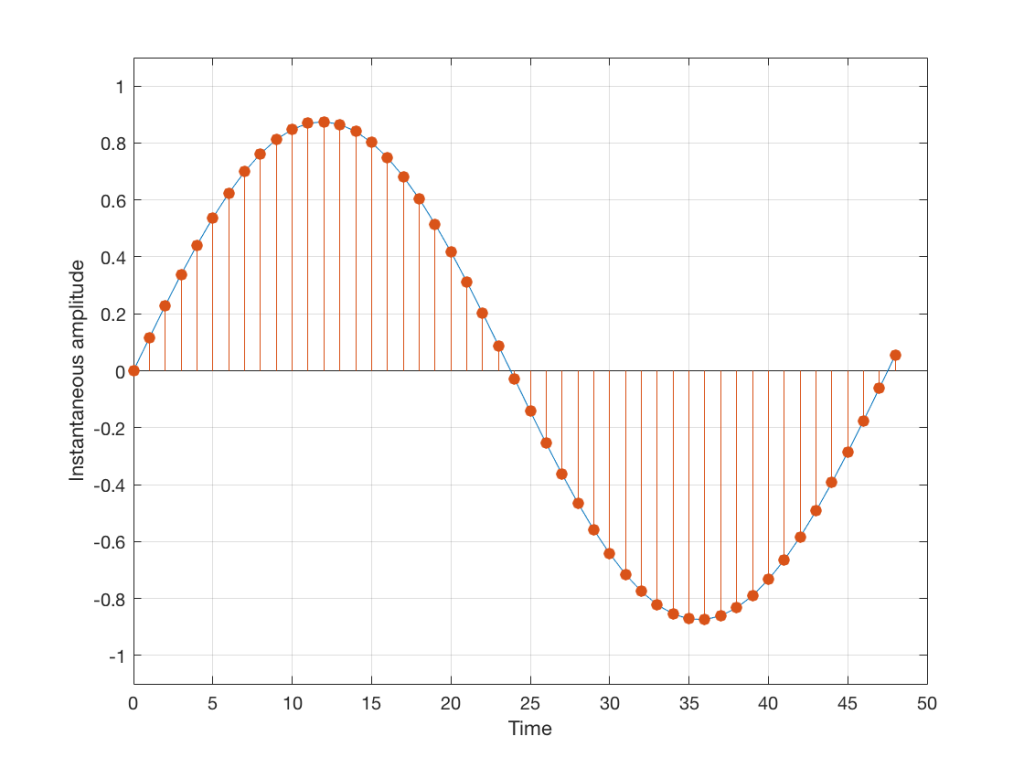Fig 2: An audio signal (blue) that has been sampled at discrete time intervals, but still has infinite resolution in its amplitude measurement (shown in red).

So, in Figure 2 we have a representation of a sinusoidal wave that has been “sampled” – a word that means “measured at regular time intervals. We are grabbing a “sample” or a “measurement” of the amplitude of the signal.

The problem is that the “ruler” we use to measure those values doesn’t have infinite resolution – just like the ruler that you would use to measure the length of something. If your ruler has lines only as fine as millimetres or 1/16th of an inch, then you cannot measure something accurately to the micrometer or to 1/64th of an inch. So, you “round off” your measurement to the nearest value on the ruler.

We do the same with audio – we have a finite number of values that we can store or transmit to represent the instantaneous amplitude of the signal, so we have to round off or “quantise” the values to the nearest value that we have. The result looks something like Figure 3: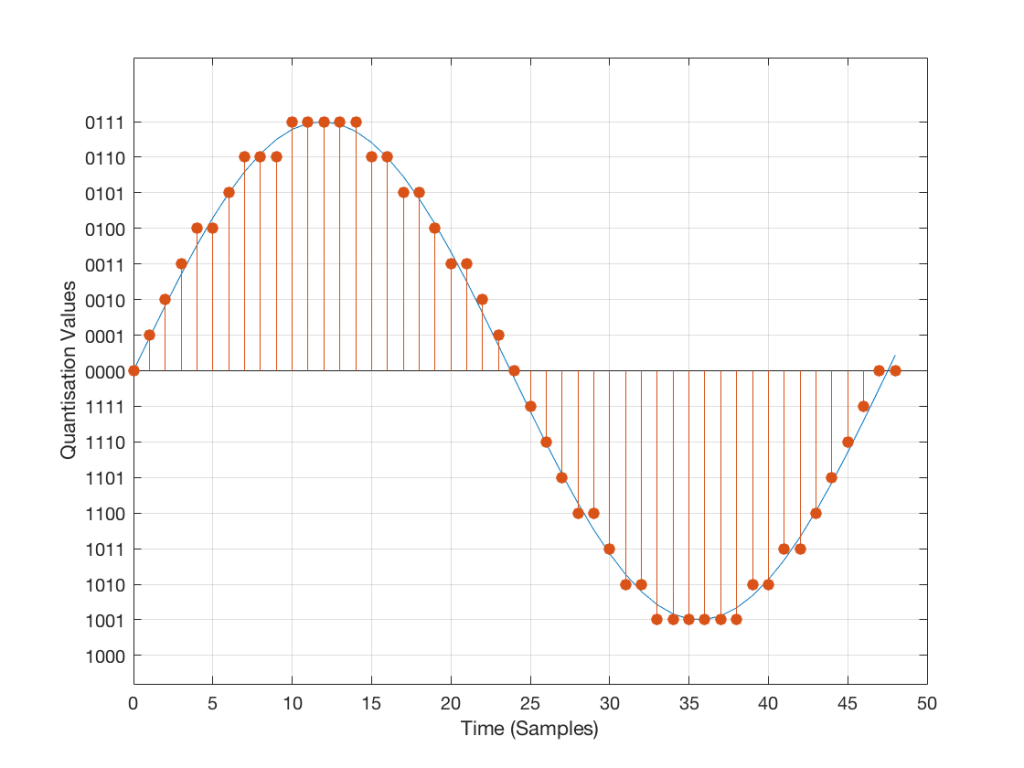Fig 3: An audio signal (blue) that has been sampled at discrete time intervals and “quantised” or “rounded off” to the nearest available amplitude value (red).

I’ve shown the quantisation values on the left (the Y-axis) as binary values. As you can see there, we have a 4-bit signal which gives us a total of 2^4 = 16 possible quantisation values for storing the signal’s amplitude at each sample.

If you’re really paying attention, you’ll notice that there are one fewer positive values than negative values, since one of the positive values is taken to represent the “0” line. This is why, when I made my original signal, I didn’t scale  it all the way up to ±1 – just to keep things smooth in the explanations. If you aren’t paying that much attention, and you didn’t notice this – then please have a look, since it will come up again later…

Normally, of course, we store audio signals with a LOT more bits than this – a CD uses 16-bit resolution, which gives us a total of 65536 possible quantisation levels (2^16). Other systems use a different number of bits – either fewer or more, depending.

At this point, it should be pretty clear that you have a finite number of samples (or measurements) per second (typically 44100 samples per second (or 44.1 kHz), if it’s a CD, although 48000 samples per second (48 kHz) is also a pretty common number – other systems use other values for this.)

So, if we look at a CD, we have 44100 samples per second, and  65536 possible quantisation values to choose from for each sample (because it’s a 44.1 kHz, 16-bit system). Notice that we have more quantisation values than samples per second…

Now, let’s say that we want to test a piece of digital audio gear, and one of the tests that we wanted to perform was to ensure that all possible quantisation values are working properly (whatever that means). Let’s also say that the gear has only 4 bits of resolution and is running at a sampling rate o 48 kHz, to start. One way to test any audio gear is to feed in a sine tone and to see what comes out. So, we’ll do that, using a 1 kHz sine tone. The result looks like Figure 4, below.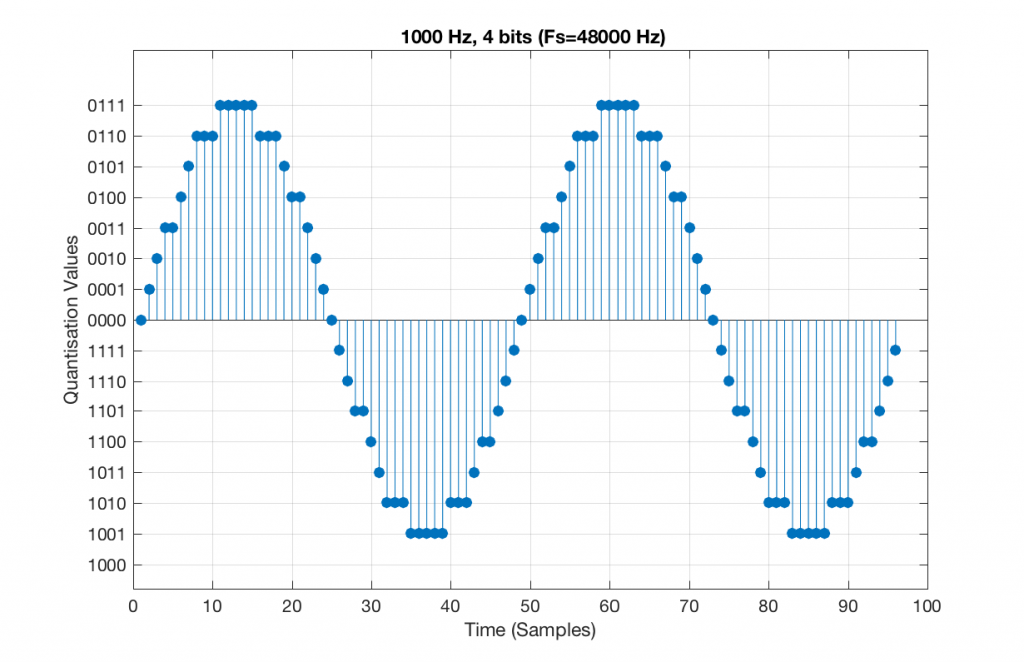Fig 5. A 1 kHz sine tone, represented in a PCM system with 4 bits of resolution and a sampling rate of 48 kHz.

There are two things to notice about that signal in Figure 5:

• The first is that all possible quantisation values are used at least once – except for the very bottom one – but that last one is my fault, caused by the scaling of the sine wave, and the fact that it is symmetrical.
• The second is that the wave is perfectly periodic – meaning that it repeats itself over and over and over… There are two cycles of the waveform shown in the plot, and if you count the dots, you’ll see that the two are identical. This second point is the one that will be important to understand as we go further. The reason this exact repetition happens is because the frequency of the sine tone (1000 Hz) is an integer divisor of the sampling rate (48000 Hz). In other words, 48000 / 1000 = 48 – not a weird number like 48.3.

Let’s take that same signal (1 kHz in a 4-bit, 48 kHz PCM system) and we’ll count the number of times each sample value occurs after 1 second (or in a time of 48000 samples). We can then plot these values as is shown in Figure 6, which is a kind of plot called a “histogram”.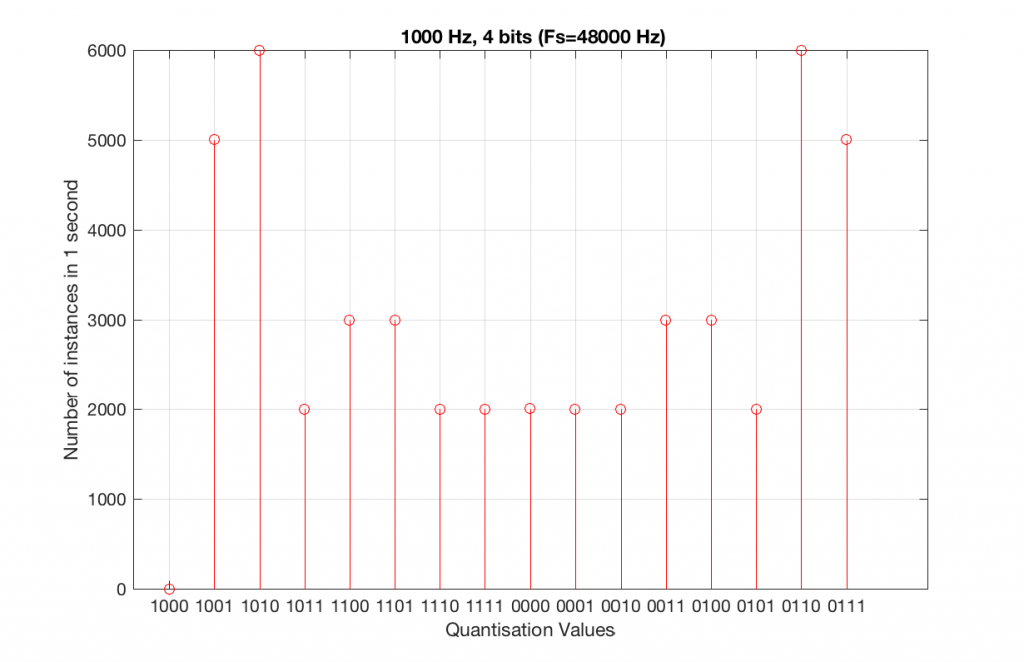Fig 6. A histogram of the number of times each quantisation value is used in 1 second of a 1 kHz sine tone in a 4-bit, 48 kHz PCM system.

As can be seen in Figure 6, the bottom quantisation value (1000) is never used – but apart from that one, all others are.

Let’s do the same thing, but with a 4-bit, 44.1 kHz system instead. The results of this are shown below in Figure 7 and 8.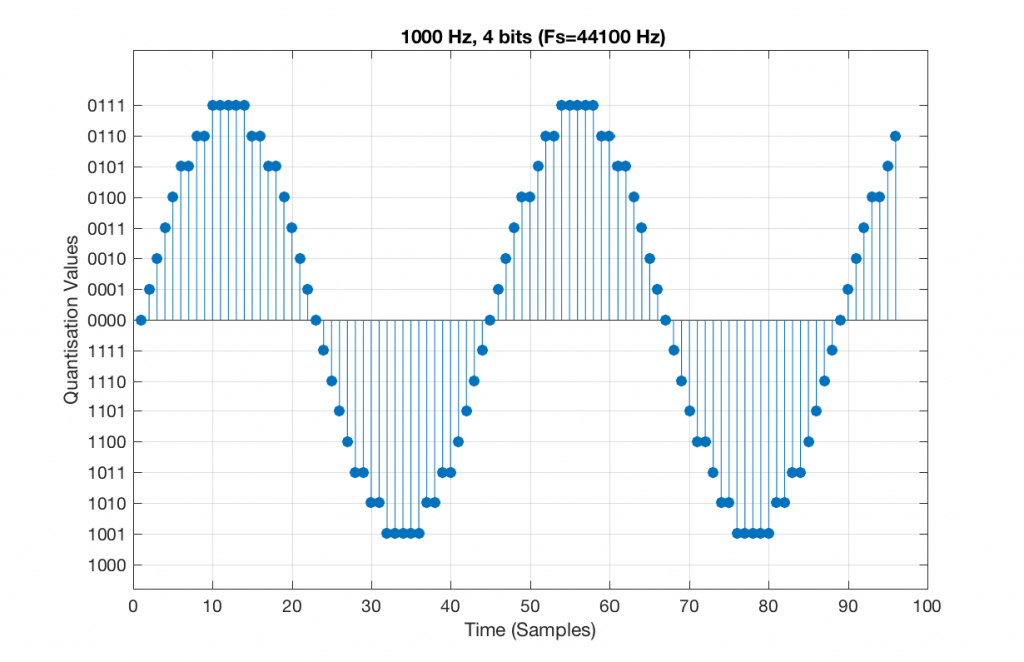Fig 7. A 1 kHz sine tone, represented in a 4-bit, 44.1 kHz system. Notice that the second instance of the waveform is not identical to the first. This is because 44100 / 1000 = 44.1 – not an integer value.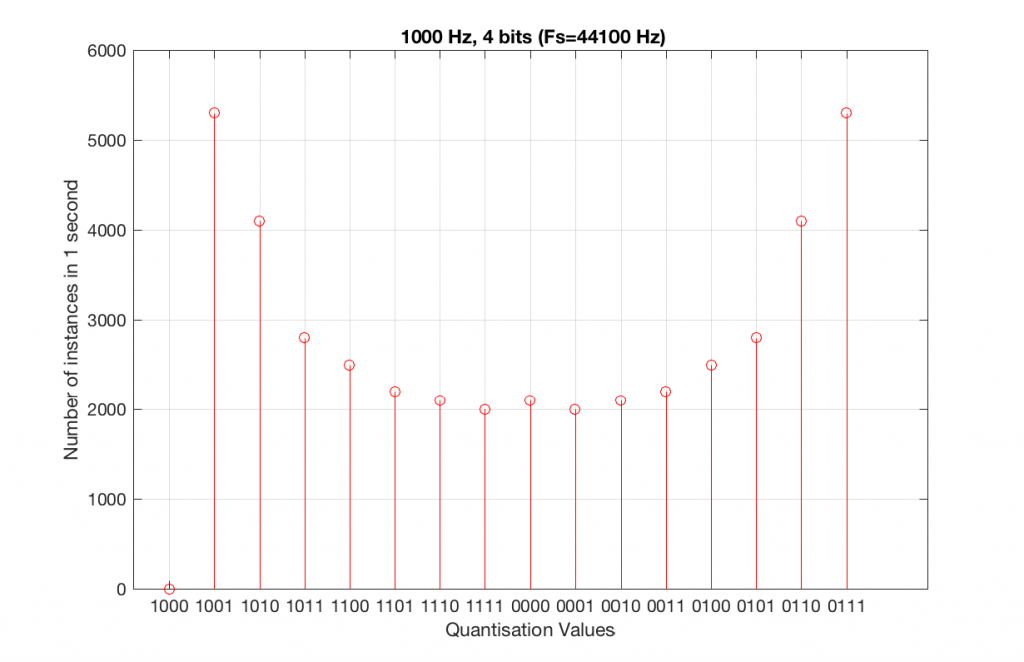Fig 8. A histogram of the quantisation values of 1 second of a 1 kHz sine tone in a 4-bit, 44.1 kHz PCM system.

Compare Figures 6 and 8. Notice that Figure 8 appears to be a “smoother” shape. This is due to the fact that the instances of the waveform are not identical copies of each other. As can be seen in Figure 7, the waveform is slightly different. Of course, after a full second, then the whole cycle repeats itself, since there are 1000 cycles per second in the signal, and 44100 samples per second. If the signal were 1000.1 Hz, then it would take 10 seconds for the repetition to start.

Let’s increase the number of bits and see what happens. We’ll take it up to 6 bits.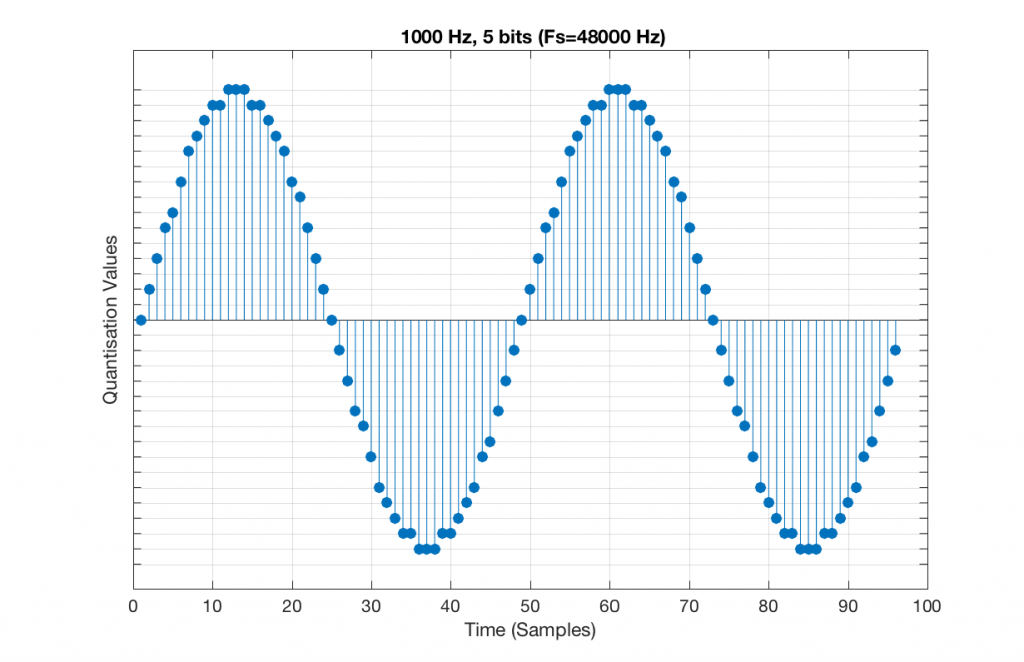Fig 9. A 1 kHz sine tone, represented in a PCM system with 5 bits of resolution and a sampling rate of 48 kHz.

Figure 9 shows a 1 kHz sine tone in a 5-bit, 48 kHz system. Again, since 48000/1000 = 48, the two cycles are identical to each other. However, something new has happened here. If you look carefully at the positive side of the sine wave, you may notice that there are 5 quantisation values that are never used. On the negative side, there are 3 unused values, as well as the very bottom one.

So, because we are in a 5-bit system, we have 2^5 = 32 possible quantisation values, but, because we are using a 1 kHz sine tone, 9 of those possible values are never used. As a result, our histogram looks like Figure 10, below.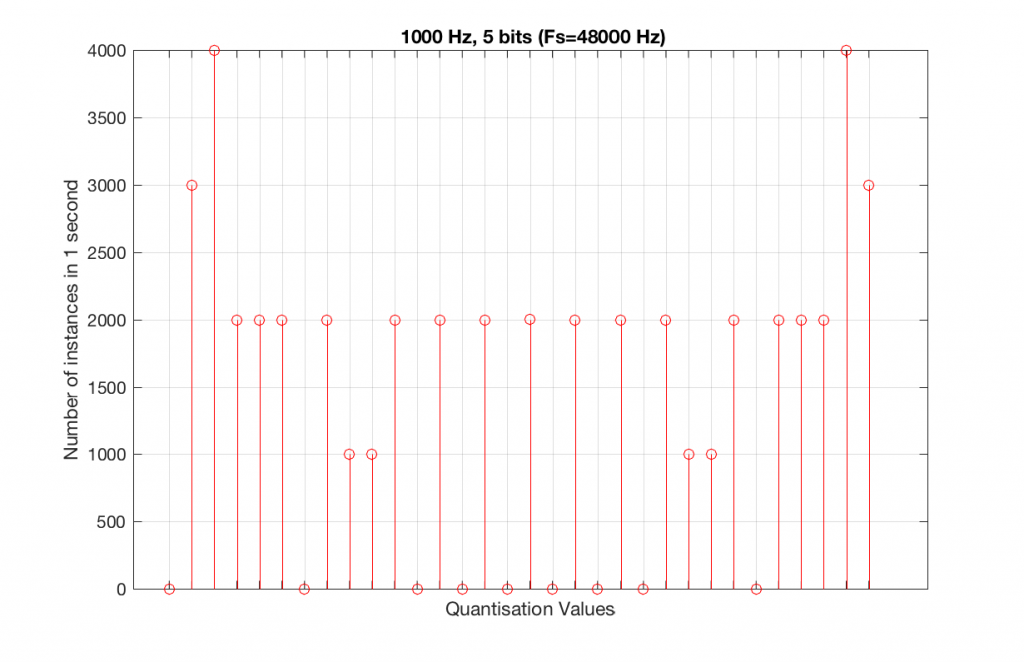Fig 10. A histogram of the quantisation values of 1 second of a 1 kHz sine tone in a 5-bit, 48 kHz PCM system. Notice that 8 of the possible 32 values are not used (plus one more at the bottom).

Let’s now compare that to a 5-bit, 44.1 kHz system.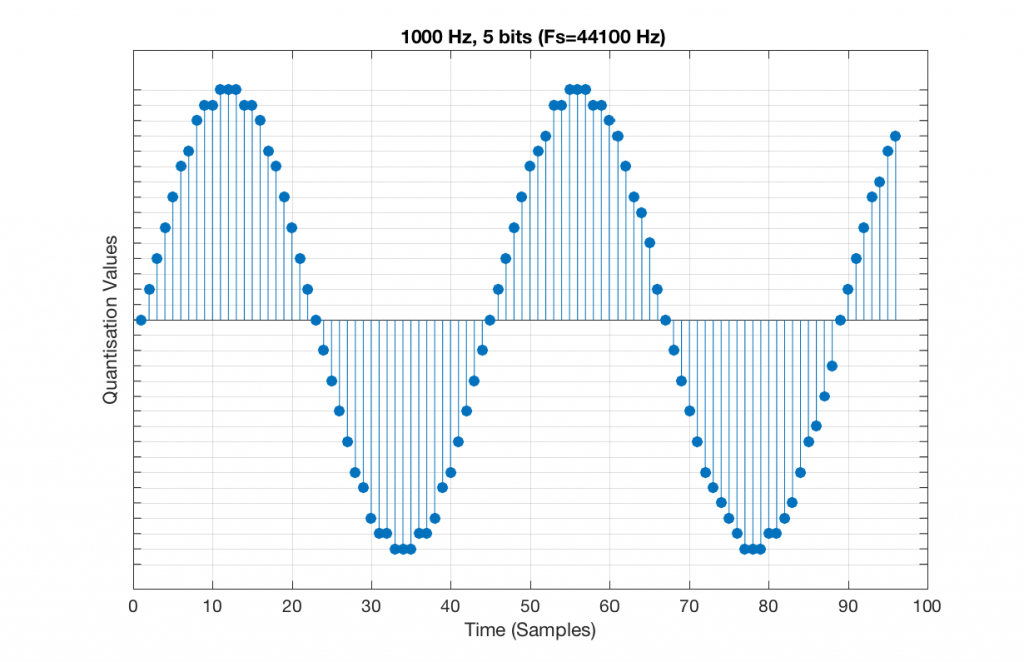Fig 11. A 1 kHz sine tone, represented in a PCM system with 5 bits of resolution and a sampling rate of 44.1 kHz.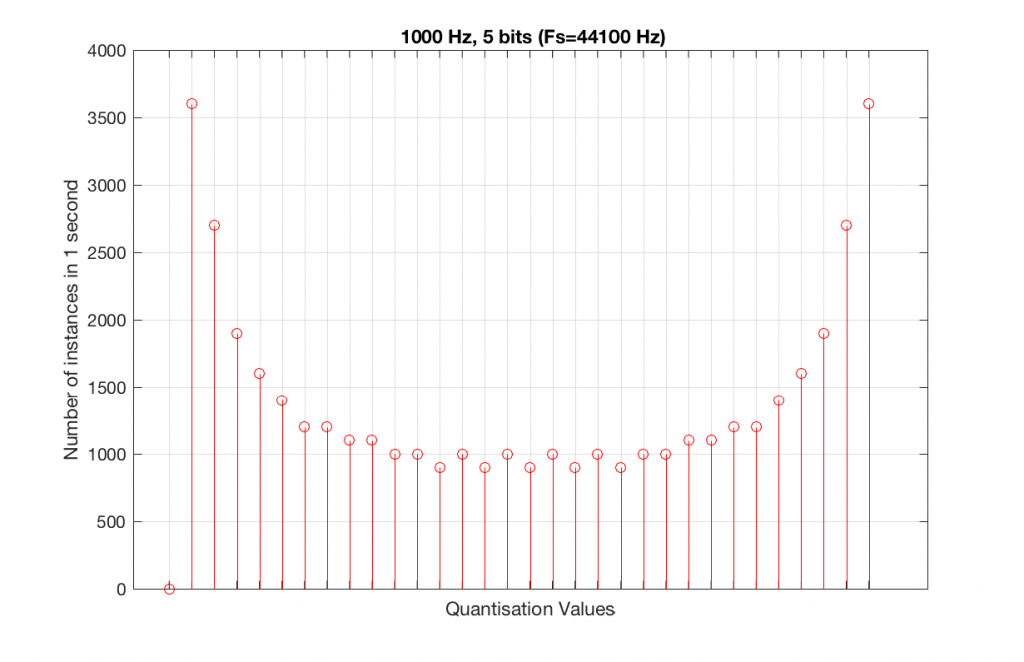Fig 12. A histogram of the quantisation values of 1 second of a 1 kHz sine tone in a 5-bit, 48 kHz PCM system. Notice that all of the possible 32 values are used (except for the bottom one…).

We can see that there is a basic problem here. The behaviour of the system may be different due only to the relationship between the sampling rate and the frequency of the signal.

The question is “what do we do about this?” We can see from Figures 10 and 12 that, when the signal’s frequency is not a nice round divisor of the sampling rate, we stand a better chance of testing the system more completely. So, instead of using a “nice” frequency like 1000 Hz, let’s use something close, but different enough to make things “misbehave” a little. One possible solution is to use 997 Hz, as we can see below: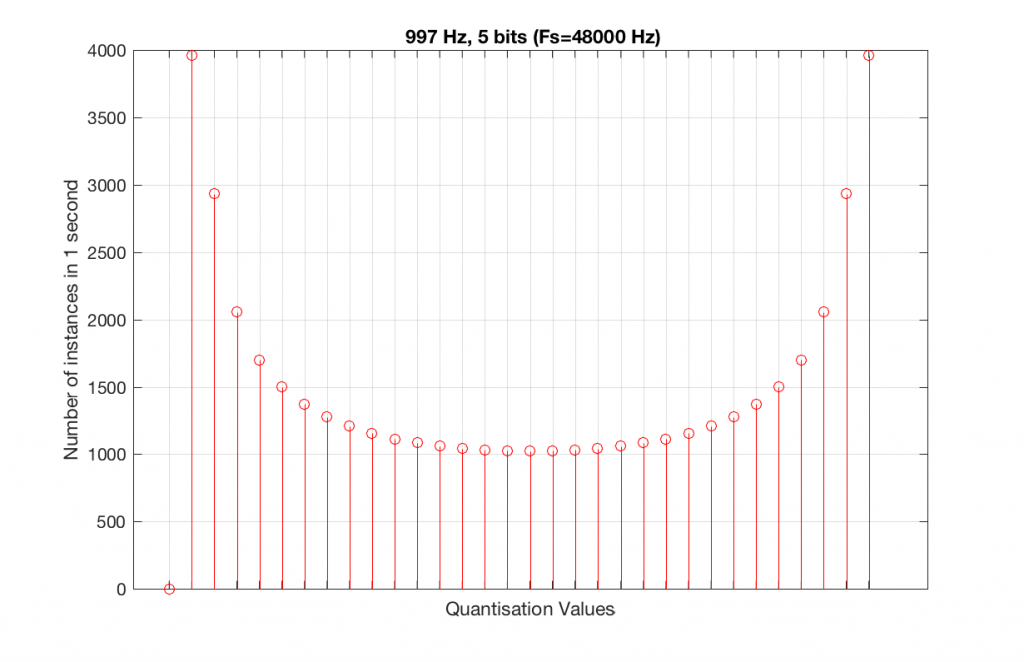Fig 13. A histogram of the quantisation values of 1 second of a 997 Hz sine tone in a 5-bit, 48 kHz PCM system. Notice that all of the possible 32 values are used (except for the bottom one…).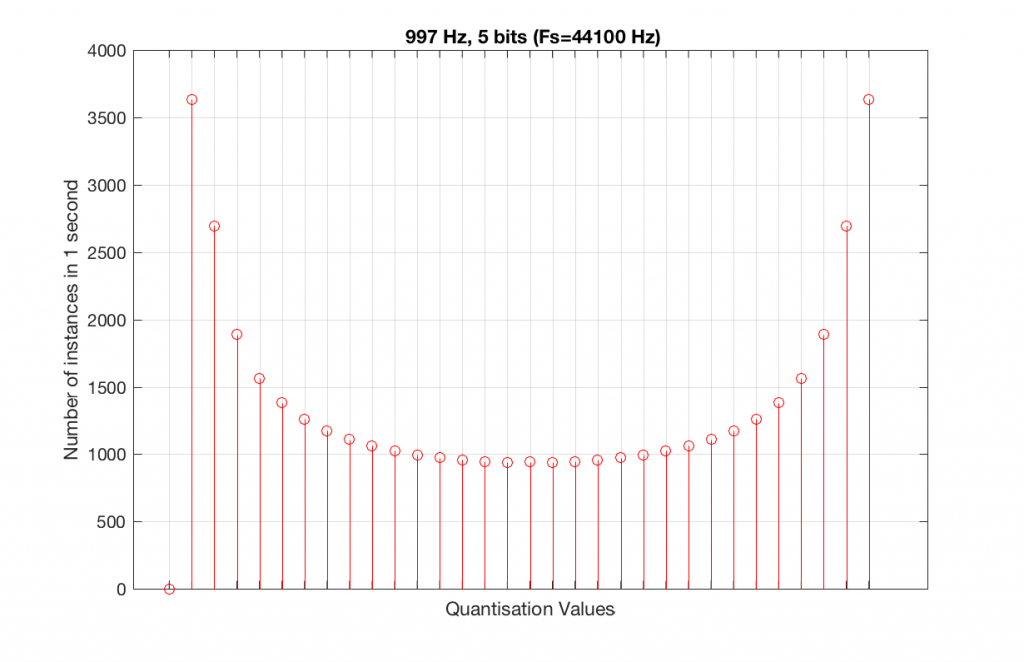Fig 14. A histogram of the quantisation values of 1 second of a 997 Hz sine tone in a 5-bit, 48 kHz PCM system. Notice that all of the possible 32 values are used (except for the bottom one…).

As can be seen in the histograms in Figure 13 and 14, changing the signal to 997 Hz from 1000 Hz results in us using all of the quantisation values in both sampling rates. So, we do a more thorough test, and stand a better chance of not missing anything…

At this point, you might say, “yes, but normally we used far more than 5 or 6 bits – this won’t happen in a system with more bits…” Nice try, but actually, things get worse, as you can see in Figures 15 and 16, below.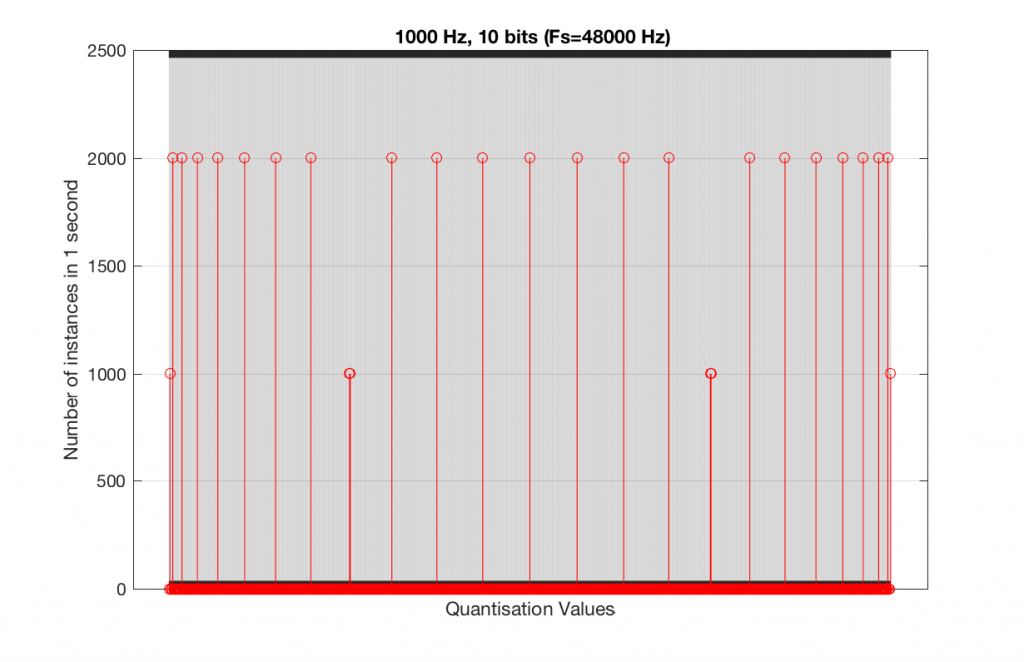Fig 15. A histogram of the quantisation values of 1 second of a 1 kHz sine tone in a 10-bit, 48 kHz PCM system.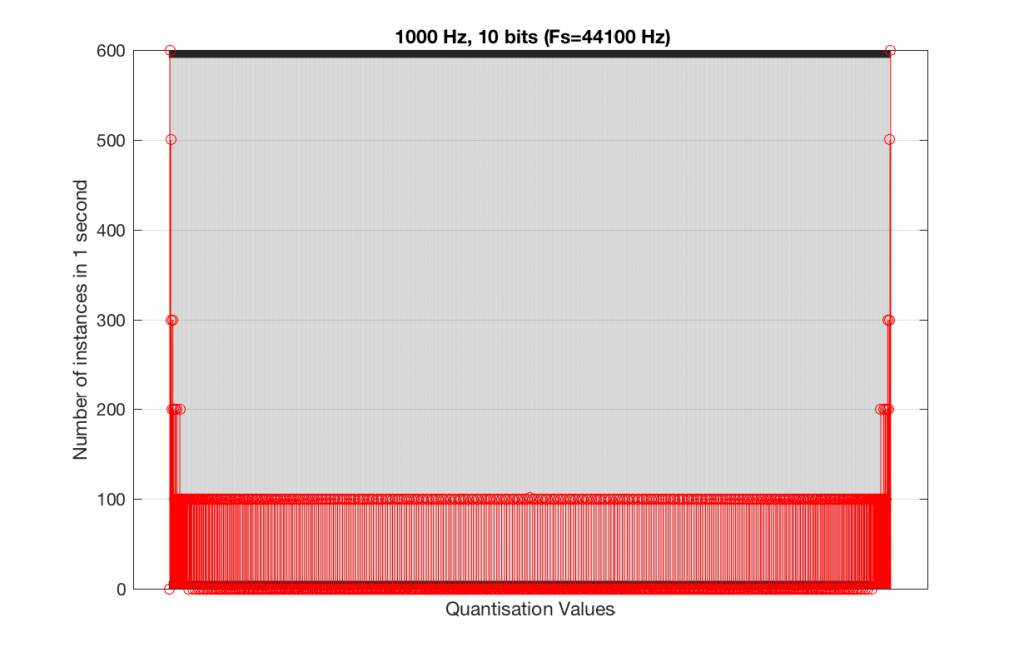Fig 16. A histogram of the quantisation values of 1 second of a 1 kHz sine tone in a 10-bit, 44.1 kHz PCM system.

As you can see in Figures 15 and 16, lots of quantisation values are unused in both sampling rates with a 1 kHz signal. By comparison, if we used a 997 Hz tone, the results would be very different, as is shown in Figures 17 and 18.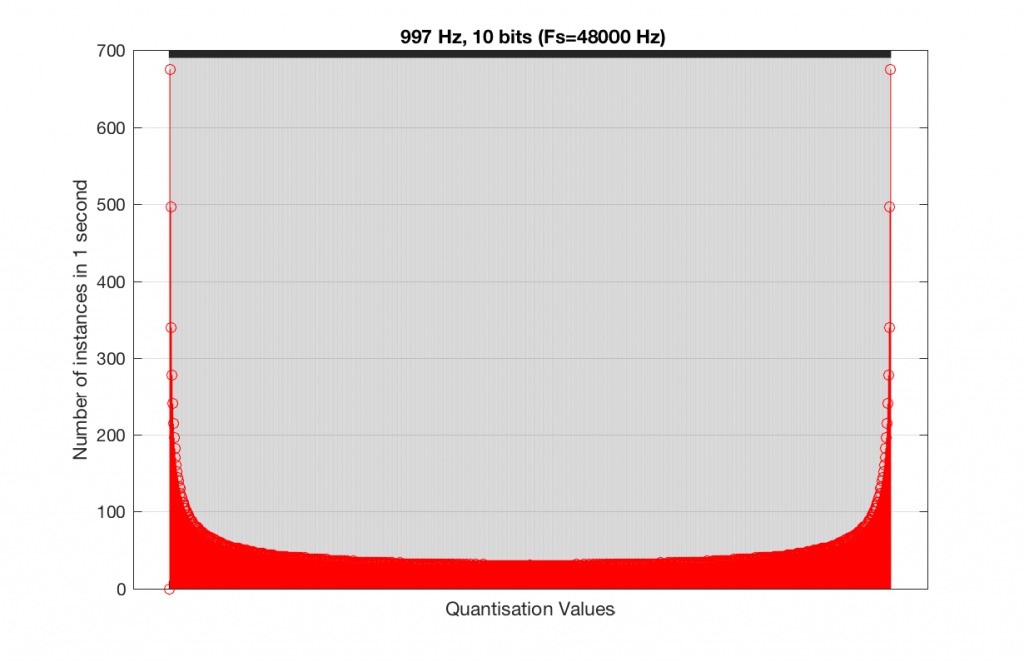Fig 15. A histogram of the quantisation values of 1 second of a 997 Hz sine tone in a 10-bit, 48 kHz PCM system.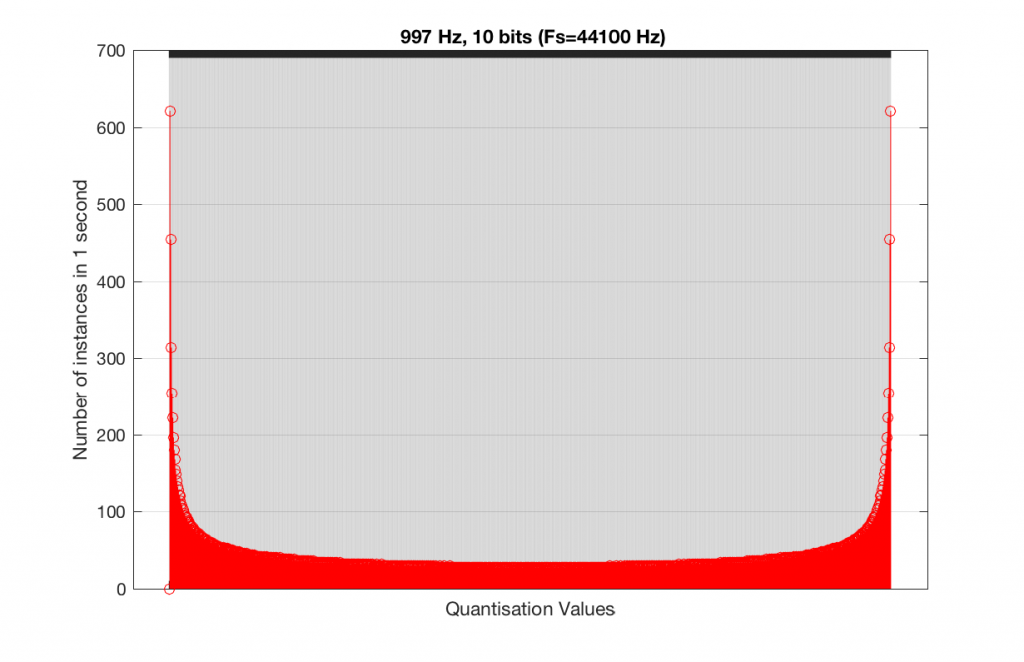Fig 16. A histogram of the quantisation values of 1 second of a 997 Hz sine tone in a 10-bit, 44.1 kHz PCM system.

In fact, as we get more and more bits of resolution, the worse the problem gets, since we have an increasing number of available of quantisation values (increasing by a factor of 2 every time we add another bit), but the number of values that we use does not increase.

This is because, at some time, we start repeating the cycle. If the sampling rate divided by the signal frequency is an integer value (like a 1 kHz tone in a 48 kHz system), then we don’t use any new quantisation values after the first cycle of the tone (or 1 ms, in this case). If the sampling rate divided by the signal frequency is not an integer value (like a 997 Hz tone in a 48 kHz system) then we don’t start repeating ourselves until 1 second has passed.

However, think back to a comment that I made up at the top – if signal does start repeating itself after 1 second (in other words, if the frequency is an integer value), and if the number of samples per second is smaller than the number of quantisation values, then we will start repeating ourselves after 1 second, and we will only test the number of quantisation values that is equal to the sampling rate.

For example, if you have a 16-bit system, then you have 65536 possible quantisation values. If the sampling rate is 48000 Hz then we could only test a maximum of 48000 possible quantisation values out of the 65536 possible ones in one second, regardless of the frequency that we choose. Typically, however, we test fewer than this, because of the repetition of some values (e.g. the maximum value, if you have a periodic signal with a frequency greater than 1 Hz).

If we do this for the two frequencies we’ve been looking at – 1 kHz and 997 Hz, for two sampling rates, 44.1 kHz and 48 kHz, at different bit depths, the results look like the following figures.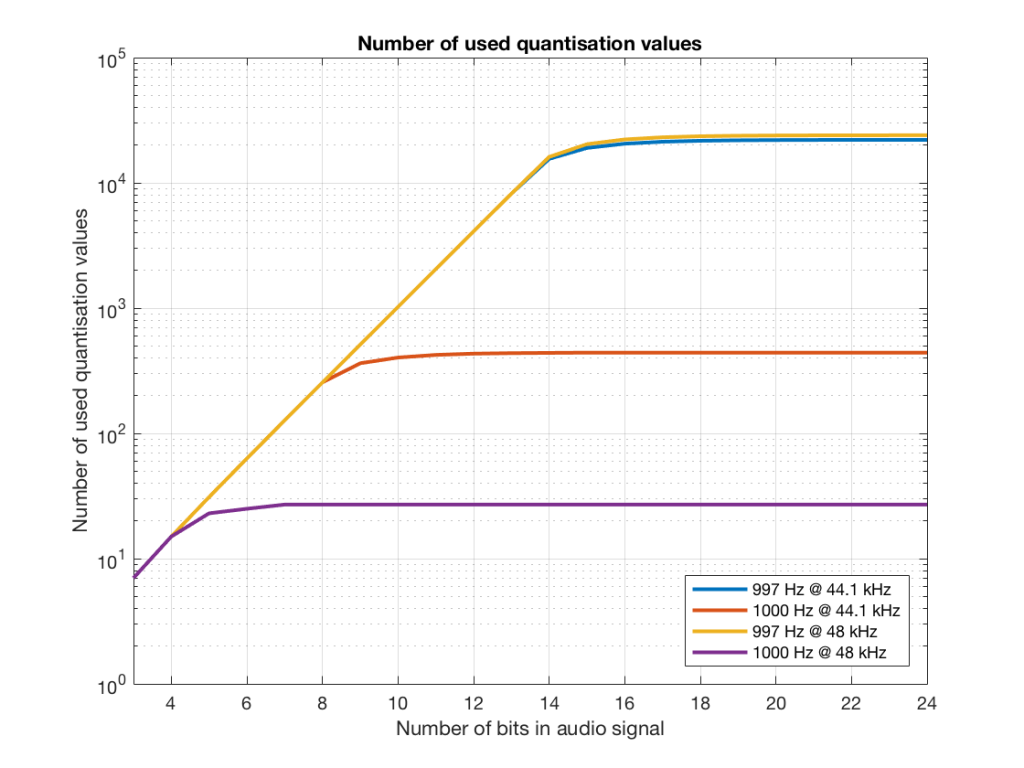Fig 17. The number of quantisation values used for 997 and 1 kHz tones in PCM systems with sampling rates of 44.1 or 48 kHz, for varying bit depths.

Notice in Figure 17 that the total number of quantisation values that are used when you have a 1 kHz tone in a 48 kHz system does not increase once you hit a word length of 7 bits. That does not mean that the signal’s representation does not improve – it does, since the quantisation values that you are using have a better resolution – so you’re rounding off less, so the error is smaller.

Notice as well that the 997 Hz tone not only results in us using far more quantisation values (topping out at the sampling rates) than the 1000 Hz tone, but that they are more similar in the two sampling rates.

If we plot the number of unused samples instead, it looks like Figure 18.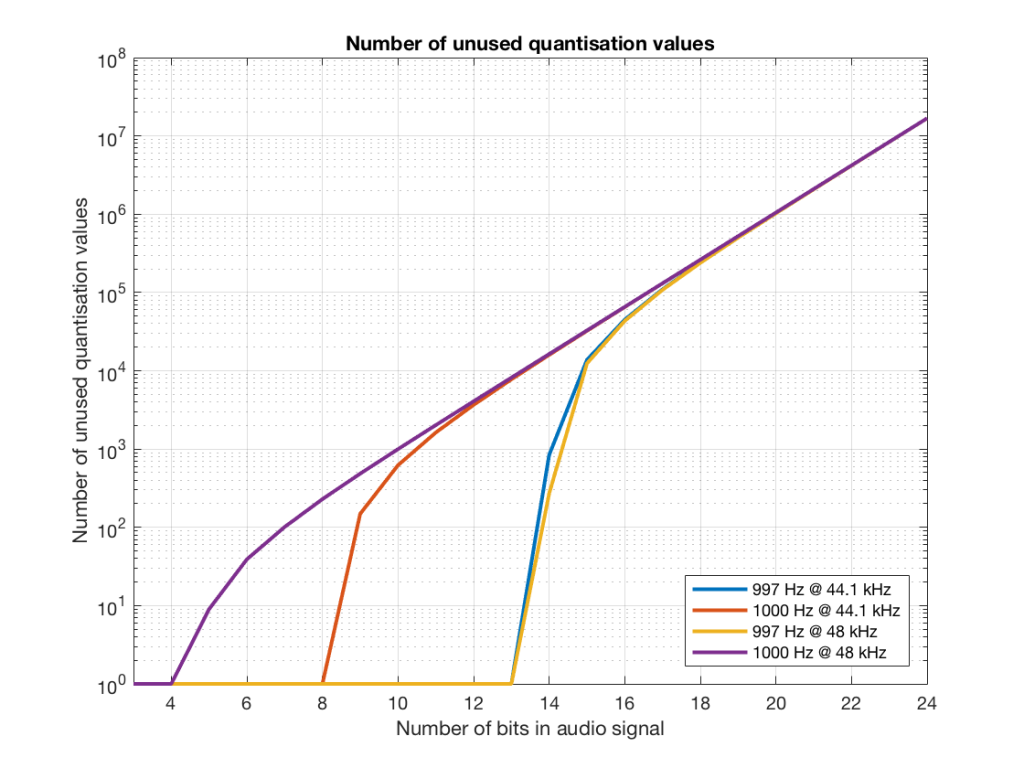Fig 18. The number of quantisation values that are not used for 997 and 1 kHz tones in PCM systems with sampling rates of 44.1 or 48 kHz, for varying bit depths.

Figure 18 is a little misleading, since as the bit depth increases, the total possible number of quantisation values also increases, however, since the two frequencies that we are analysing are integer values, the maximum number cannot go past the sampling rate. So, in an extreme case (if you choose your frequency or signal carefully), only 48000 values out of a possible 16777216 values are used in a 24-bit system per second in a system with a sampling rate of 48 kHz.

Figure 19 shows the same information as Figure 18, except that I’ve displayed the values in percent.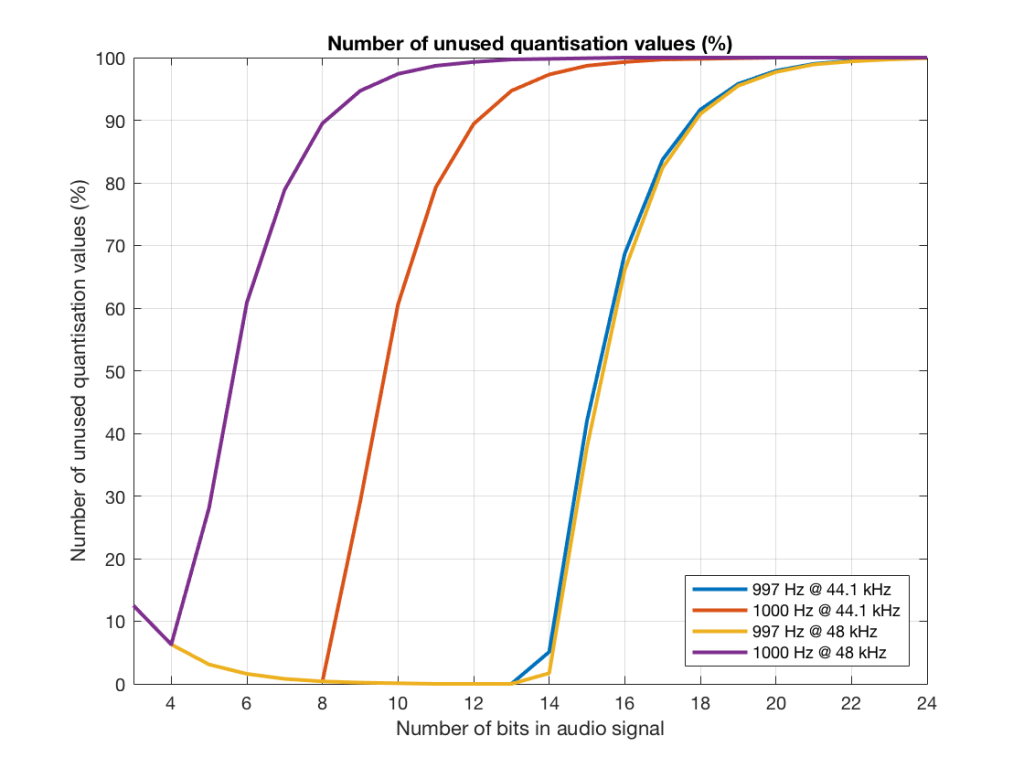Fig 19. The percentage of quantisation values that are not used for 997 and 1 kHz tones in PCM systems with sampling rates of 44.1 or 48 kHz, for varying bit depths.

So, as you can see there, in a 16-bit system, even if you use a 997 Hz tone, about 70% of the total possible quantisation values are used.

Caveat

Of course, the signals that I used here were generated digitally, and did not include dither. If I had included proper dithering, then more of the quantisation values would have been used. However, the point of this posting was not to talk about correct ways of creating PCM signals – it was an attempt to explain why we use 997 Hz instead of 1 kHz when we test digital audio systems.